# Visually explore Probability Distributions with vistributions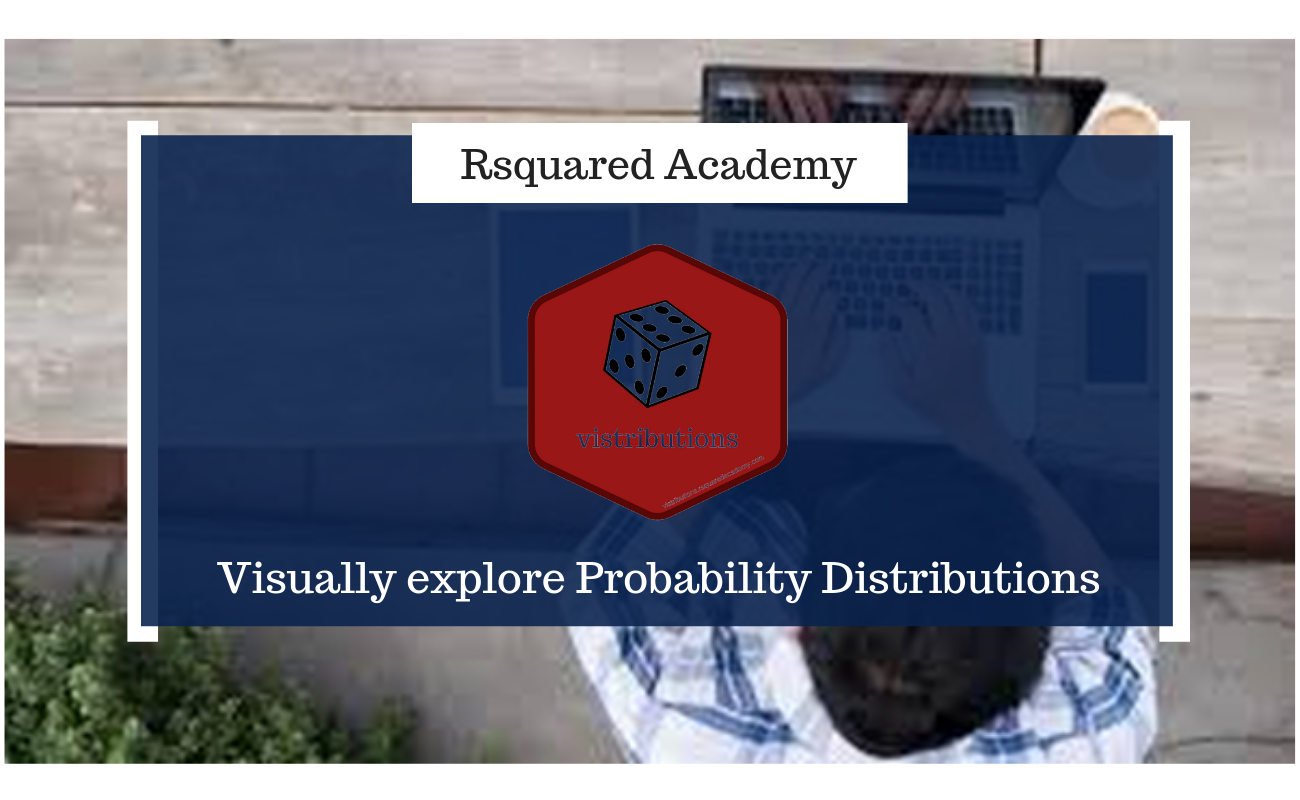We are happy to introduce the vistributions package, a set of tools for visually exploring probability distributions.

## Installation

``````# Install release version from CRAN
install.packages("vistributions")

# Install development version from GitHub
# install.packages("devtools")

## Shiny App

vistributions includes a shiny app which can be launched using

``vdist_launch_app()``

or try the live version here.

## Tab Completion

The common `vdist_` prefix will trigger autocomplete, allowing you to see all vistributions functions: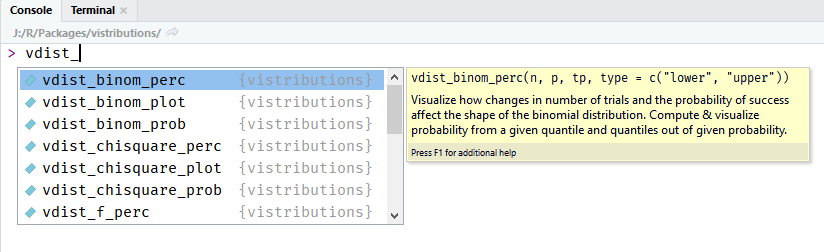In exploring statistical distributions, we focus on the following:

• what influences the shape of a distribution
• calculate probability from a given quantile
• calculate quantiles out of given probability

for the following distributions:

• Normal
• Binomial
• Chi Square
• F
• t

## Normal Distribution

### Distribution Shape

Visualize how changes in mean and standard deviation affect the shape of the normal distribution.

#### Input

• mean: mean of the normal distribution
• sd: standard deviation of the normal distribution
``vdist_normal_plot(mean = 2, sd = 0.6)``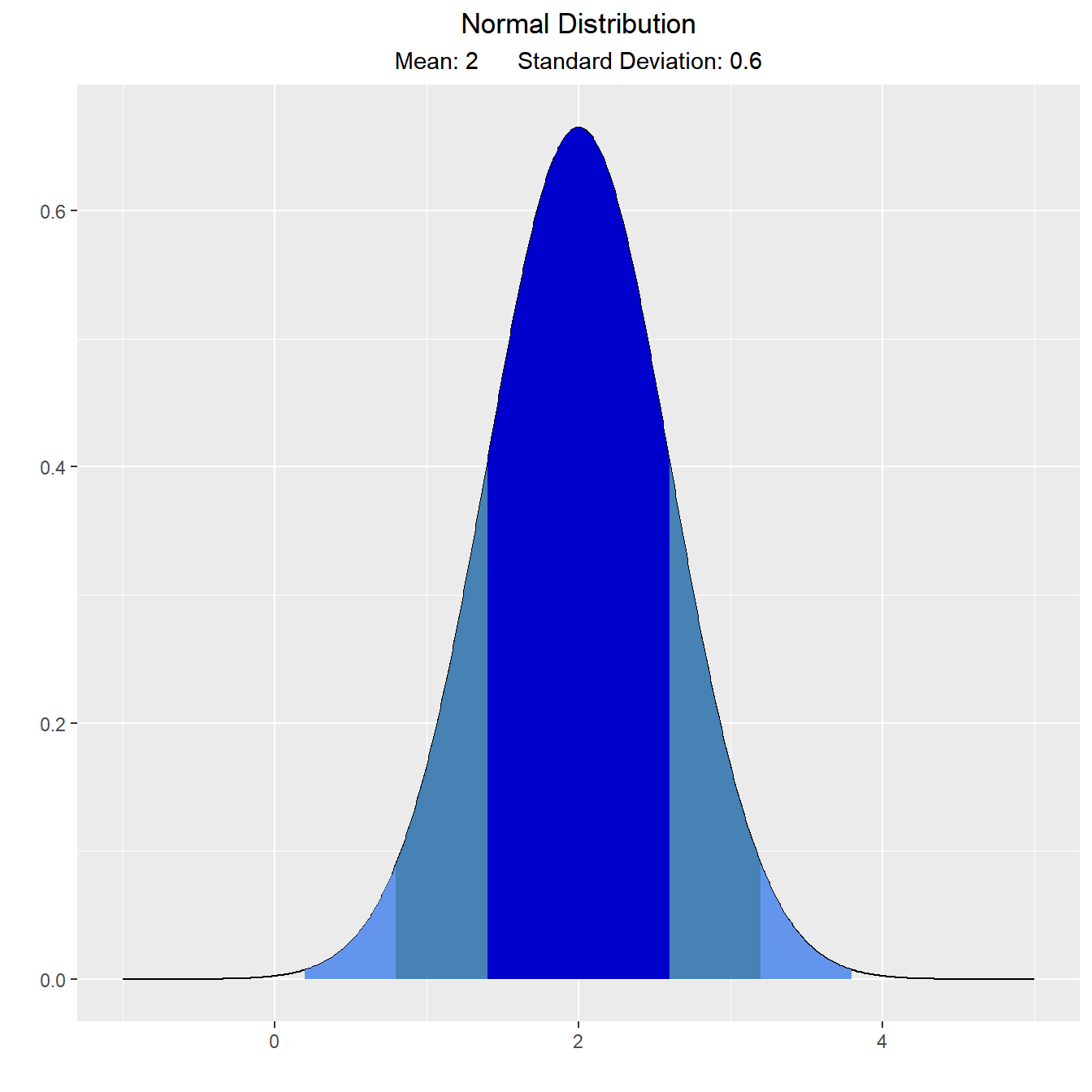### Percentiles

#### Calculate and visualize quantiles out of given probability.

##### Input
• probs: a probability value
• mean: mean of the normal distribution
• sd: standard deviation of the normal distribution
• type: lower/upper tail

Suppose X, the grade on a exam, is normally distributed with mean 60 and standard deviation 3. The teacher wants to give 10% of the class an A. What should be the cutoff to determine who gets an A?

``vdist_normal_perc(0.10, 60, 3, 'upper')``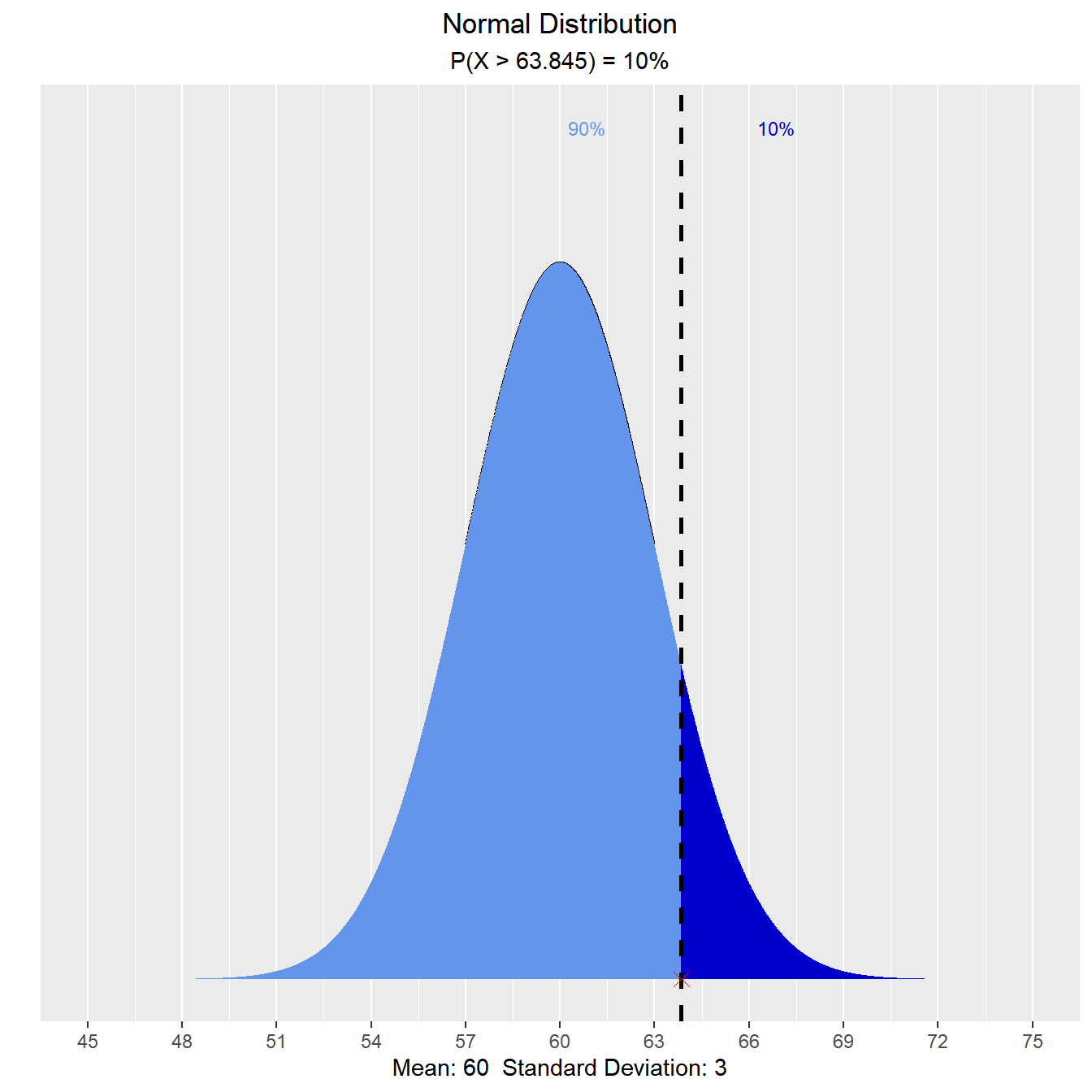The teacher wants to give lower 15% of the class a D. What cutoff should the teacher use to determine who gets an D?

``vdist_normal_perc(0.85, 60, 3, 'lower')``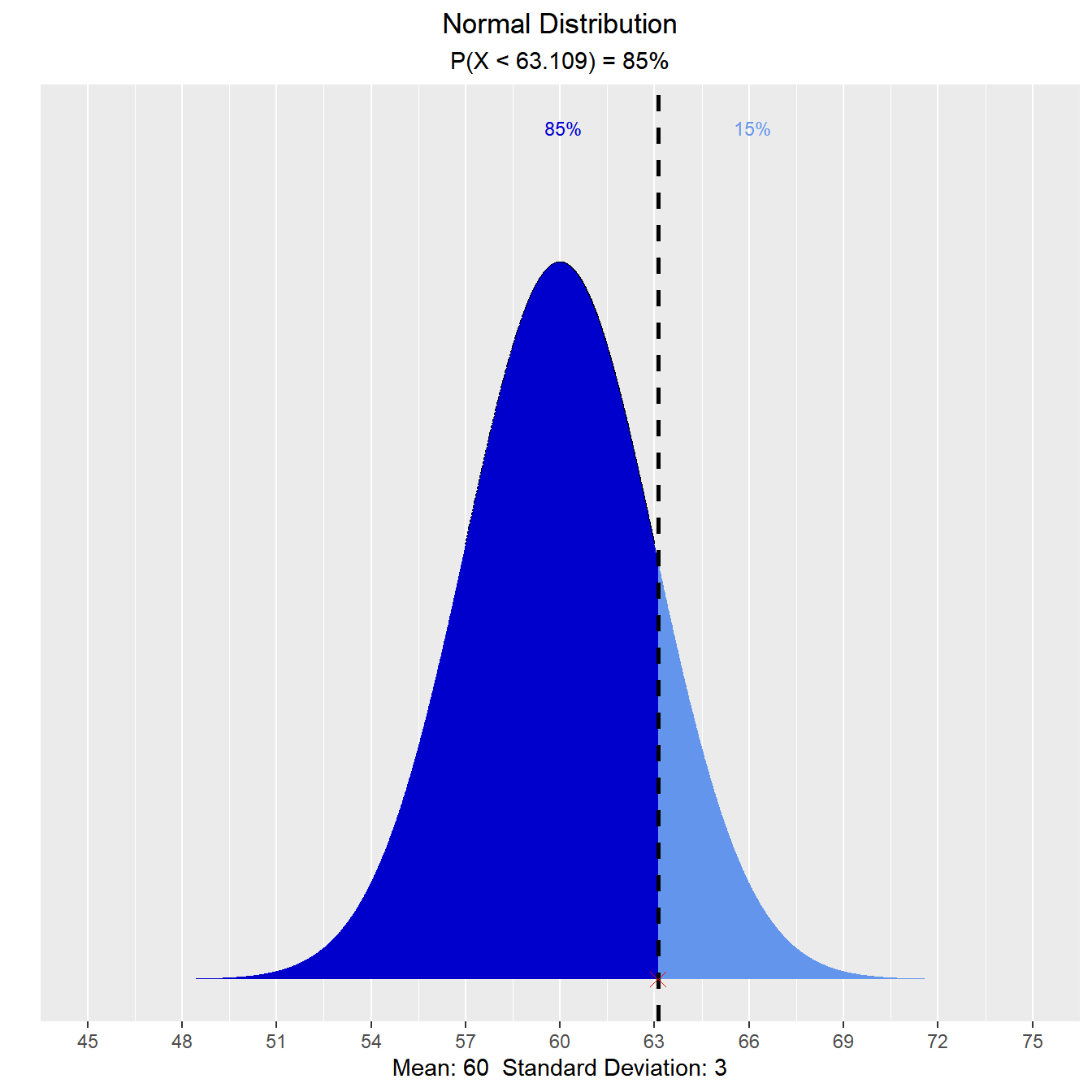The teacher wants to give middle 50% of the class a B. What cutoff should the teacher use to determine who gets an B?

``vdist_normal_perc(0.5, 60, 3, 'both')``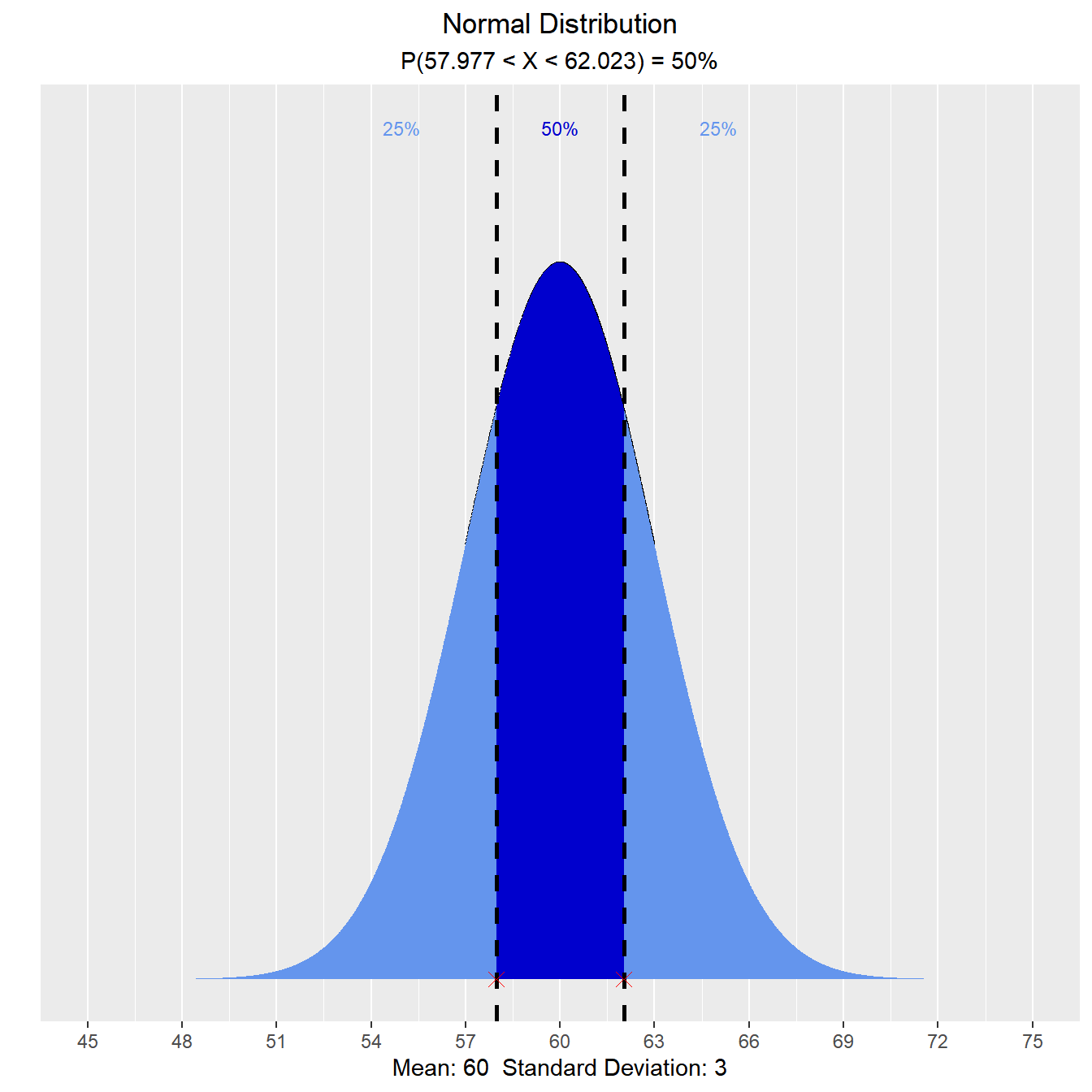### Probabilities

#### Calculate and visualize probability from a given quantile

##### Input
• perc: a quantile value
• mean: mean of the normal distribution
• sd: standard deviation of the normal distribution
• type: lower/upper/both tail

Let X be the IQ of a randomly selected student of a school. Assume X ~ N(90, 4). What is the probability that a randomly selected student has an IQ below 80?

``vdist_normal_prob(80, mean = 90, sd = 4)``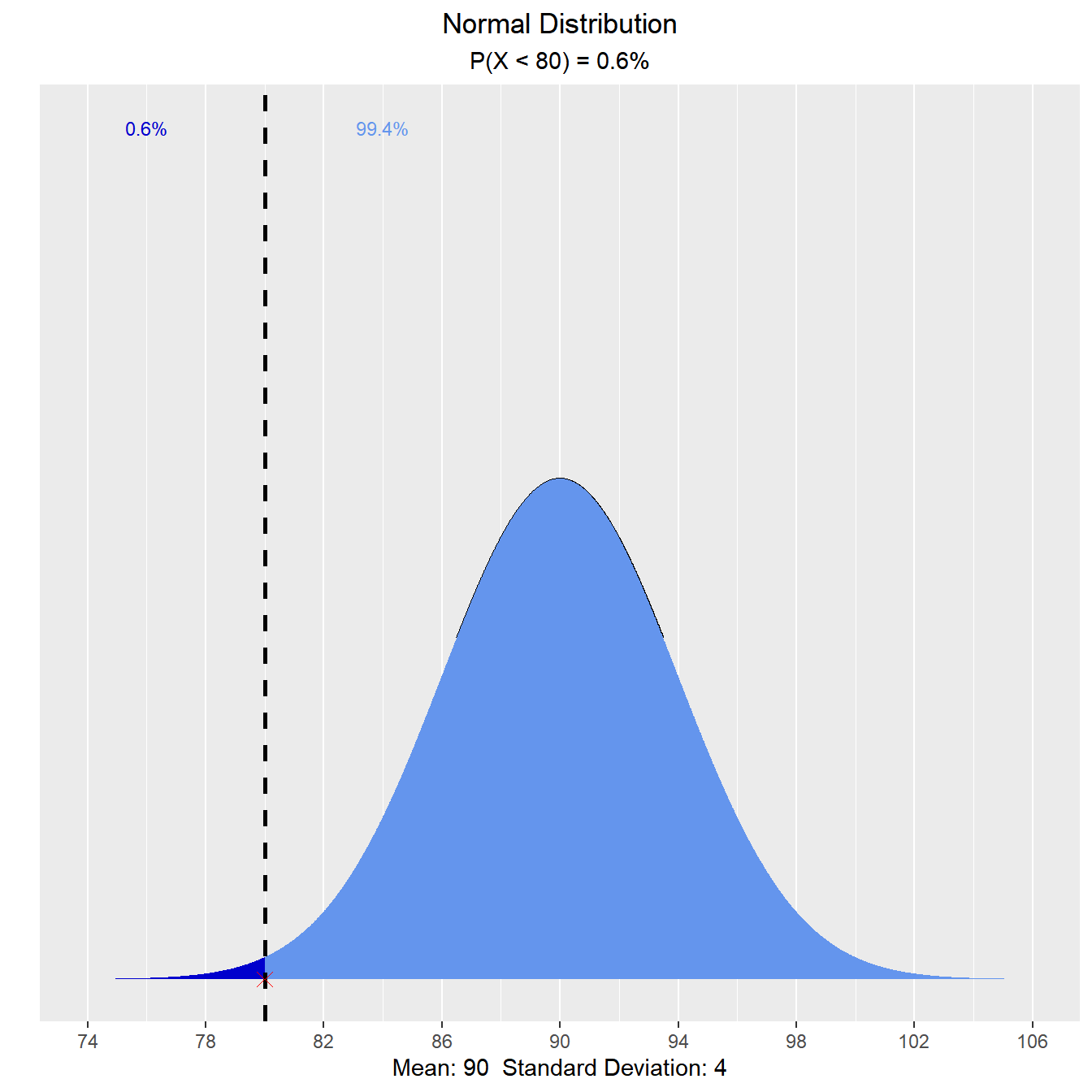What is the probability that a randomly selected student has an IQ above 100?

``vdist_normal_prob(100, mean = 90, sd = 4, type = 'upper')``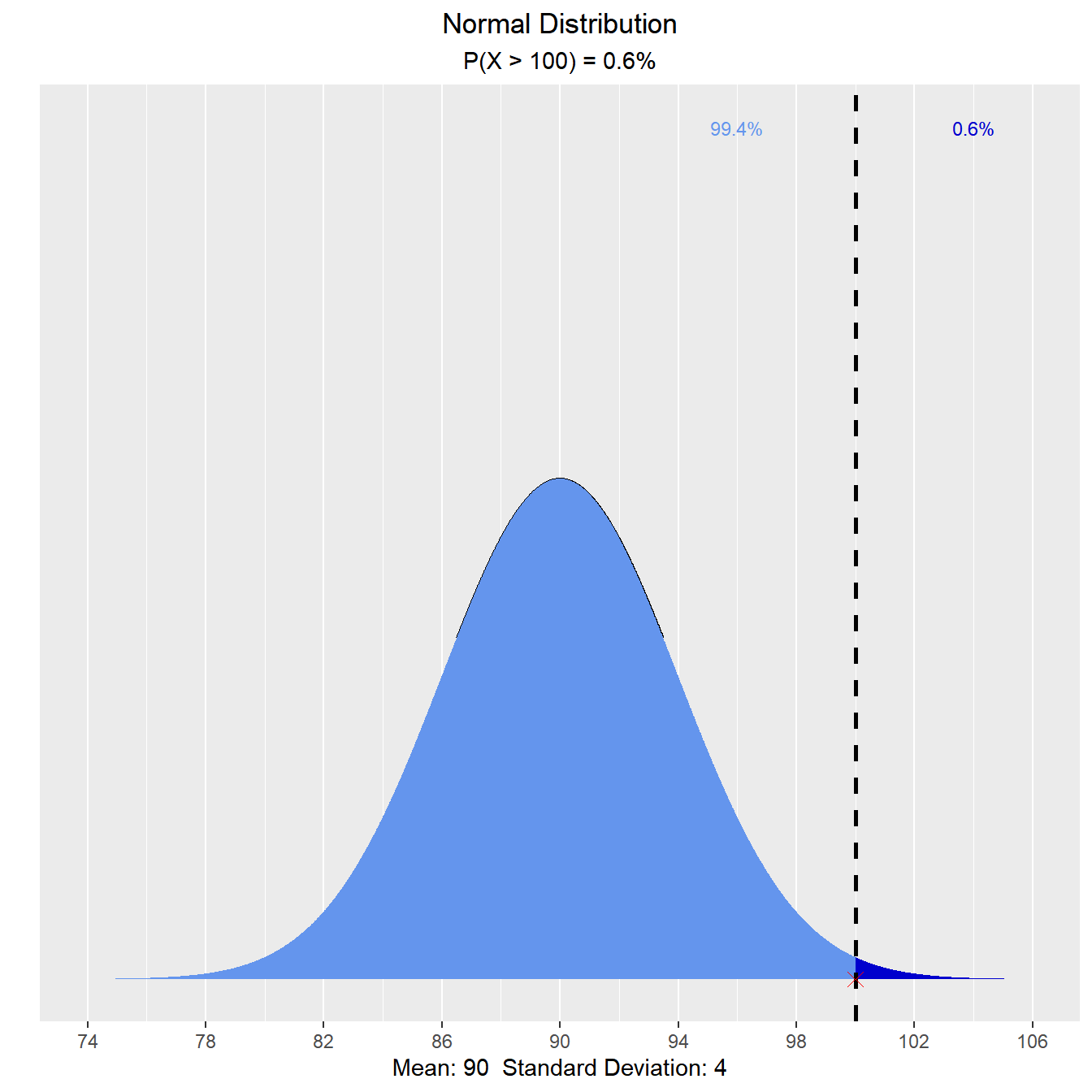What is the probability that a randomly selected student has an IQ between 85 and 100?

``vdist_normal_prob(c(85, 100), mean = 90, sd = 4, type = 'both')``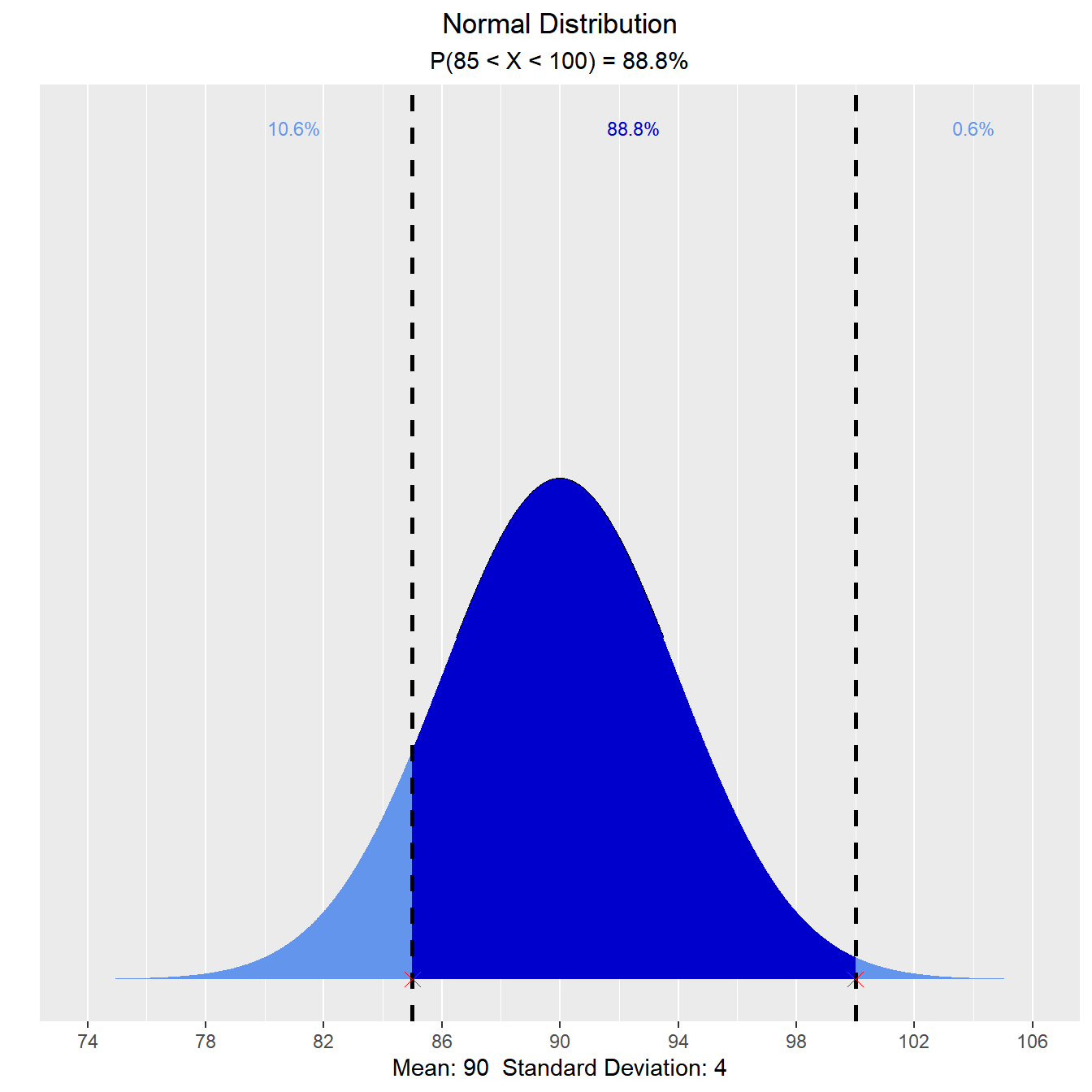## Binomial Distribution

### Distribution Shape

Visualize how changes in number of trials and the probability of success affect the shape of the binomial distribution.

``vdist_binom_plot(10, 0.3)``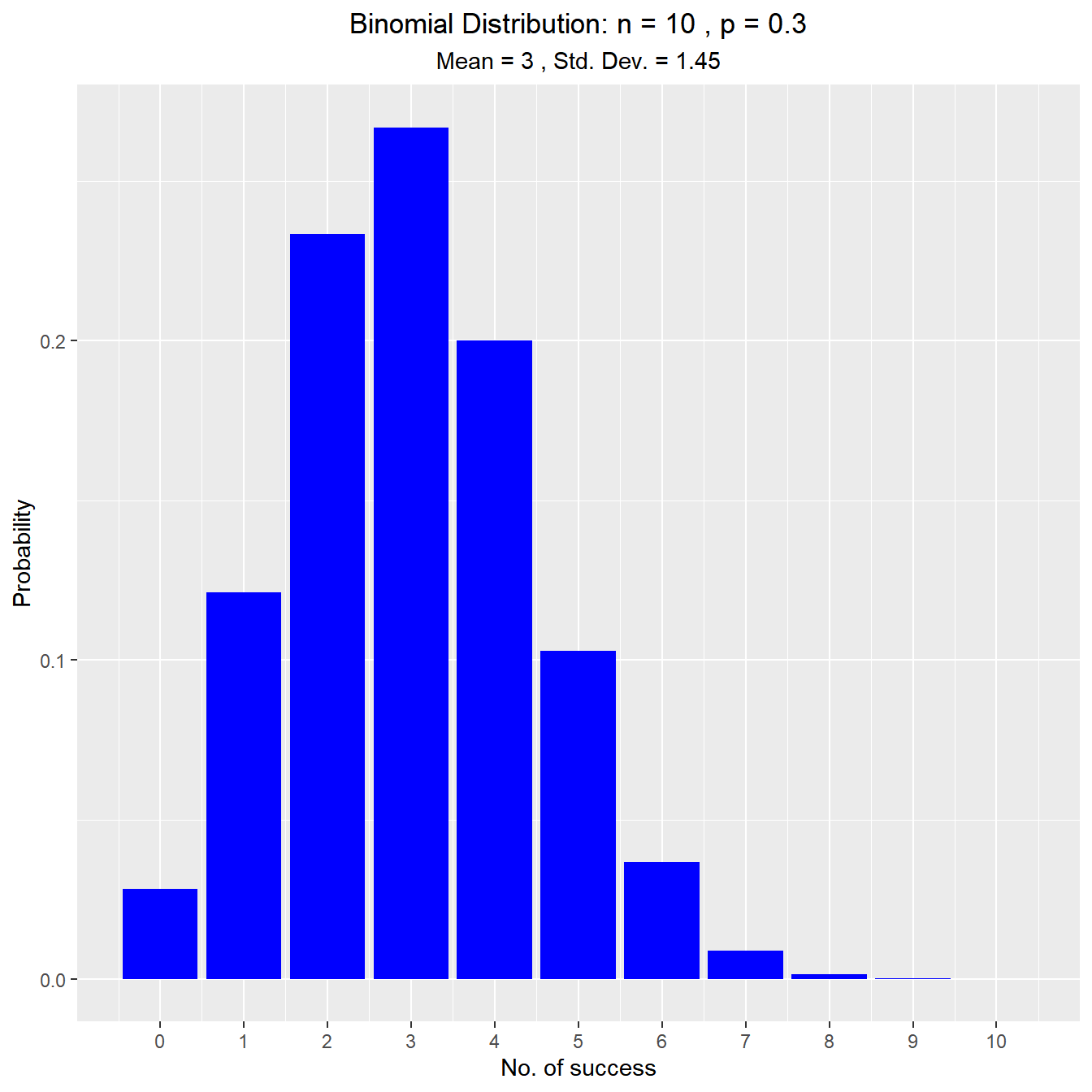### Percentiles

#### Calculate and visualize quantiles out of given probability

##### Input
• p: a single aggregated probability of multiple trials
• n: the number of trials
• tp: the probability of success in a trial
• type: lower/upper tail
``vdist_binom_perc(10, 0.5, 0.05)``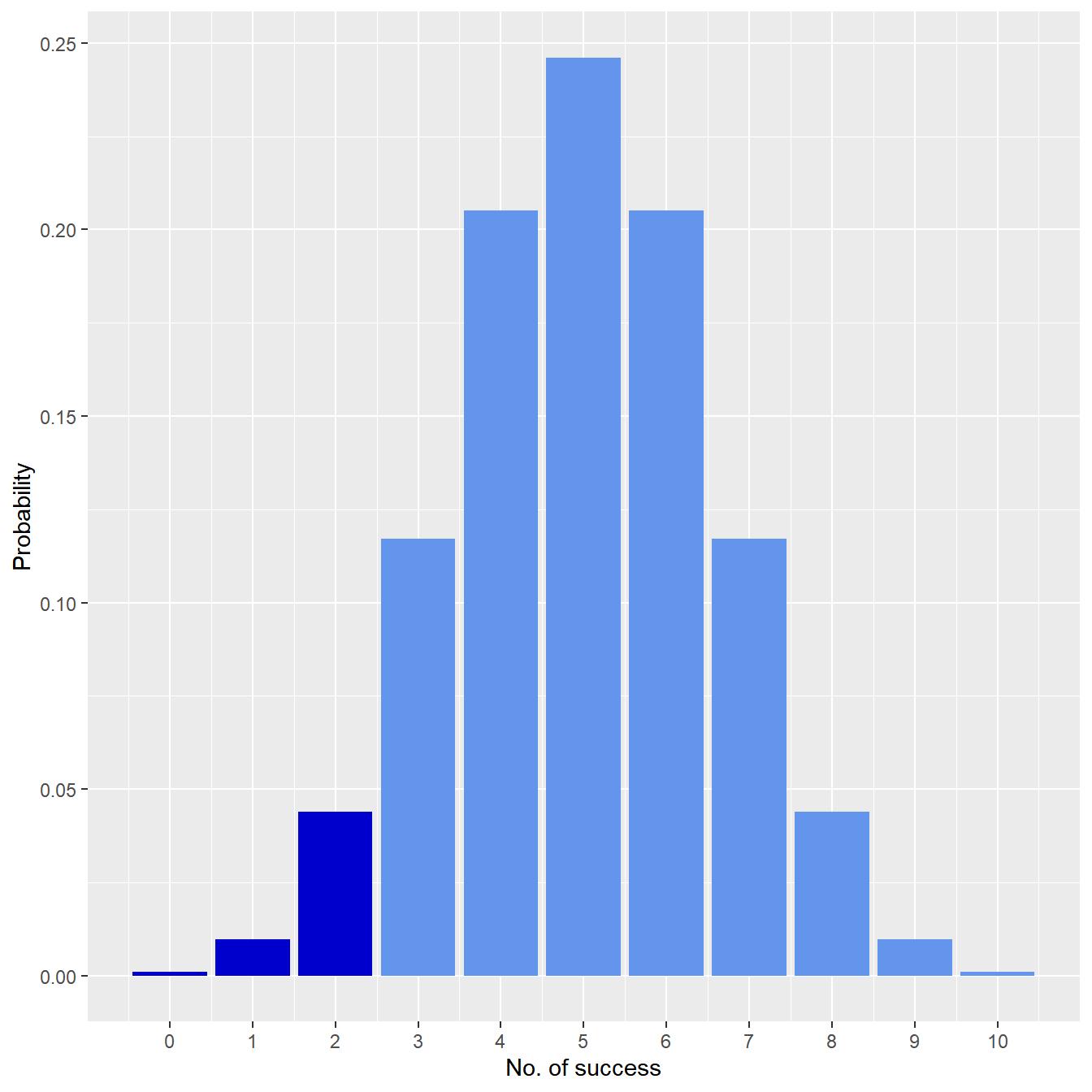``vdist_binom_perc(10, 0.5, 0.05, 'upper')``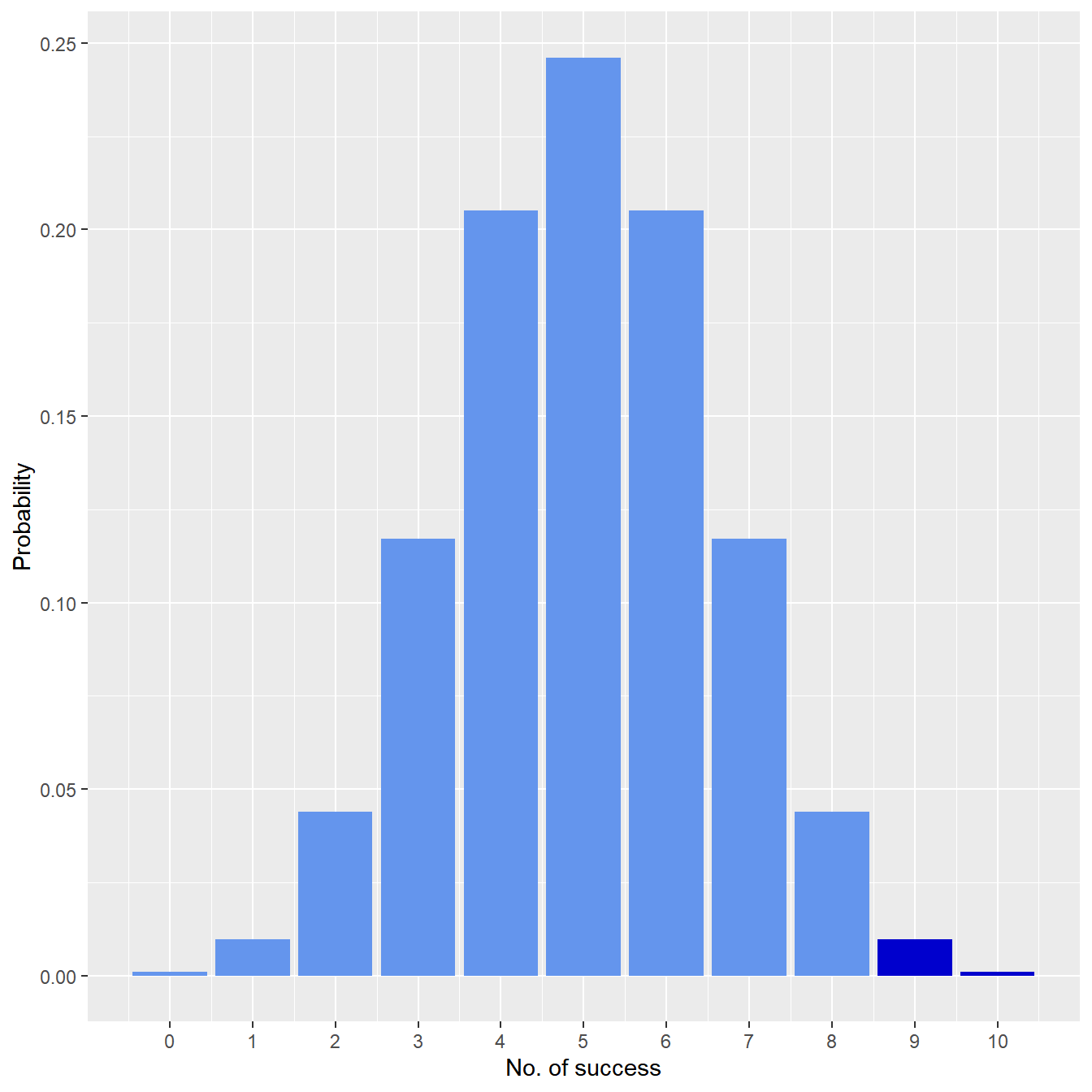### Probabilities

#### Calculate and visualize probability from a given quantile

##### Input
• p: probability of success
• n: the number of trials
• s: number of success in a trial
• type: lower/upper/interval/exact tail

Assume twenty-percent (20%) of Magemill have no health insurance. Randomly sample n = 12 Magemillians. Let X denote the number in the sample with no health insurance. What is the probability that exactly 4 of the 15 sampled have no health insurance?

``vdist_binom_prob(12, 0.2, 4, type = 'exact')``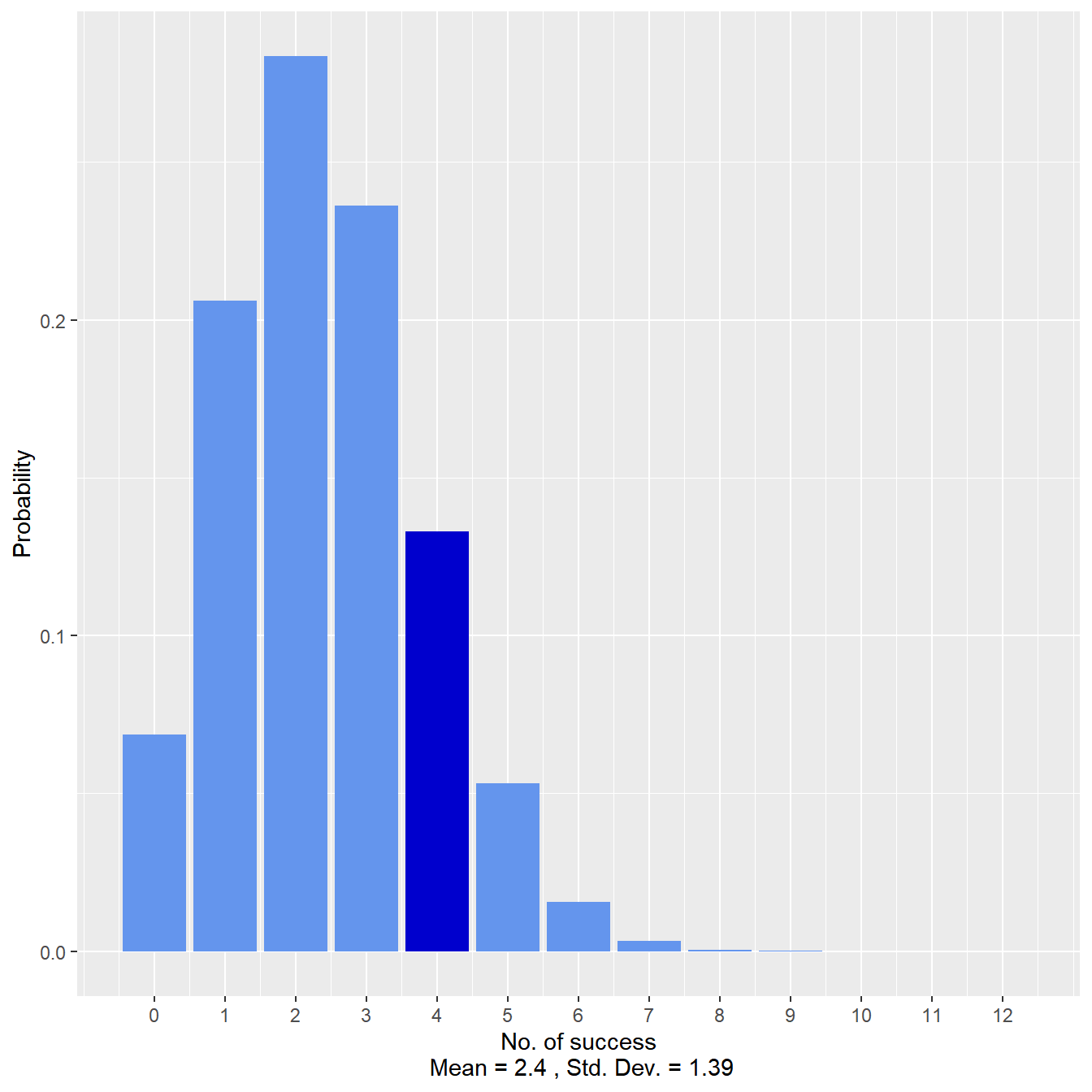What is the probability that at most one of those sampled has no health insurance?

``vdist_binom_prob(12, 0.2, 1, 'lower')``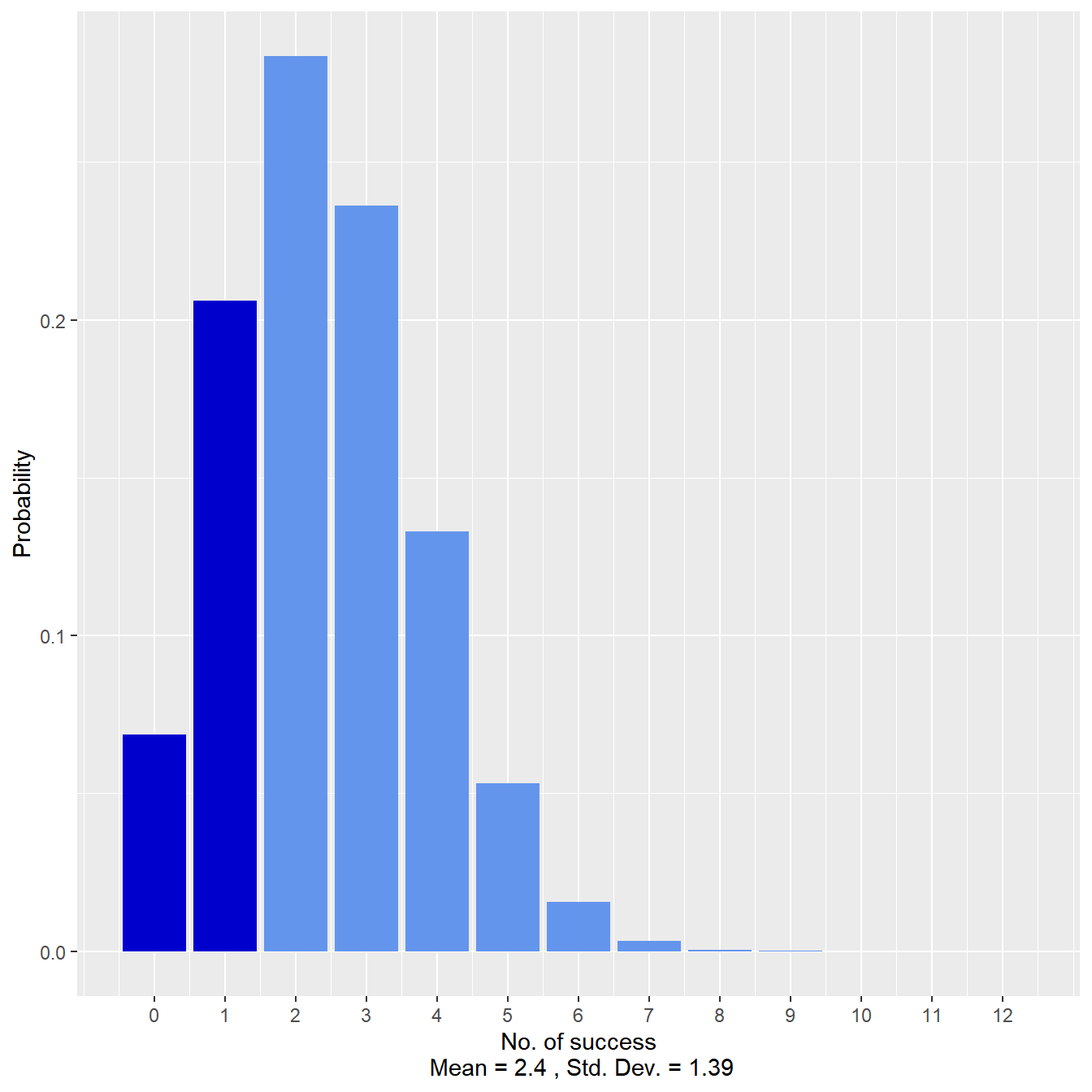What is the probability that more than seven have no health insurance?

``vdist_binom_prob(12, 0.2, 8, 'upper')``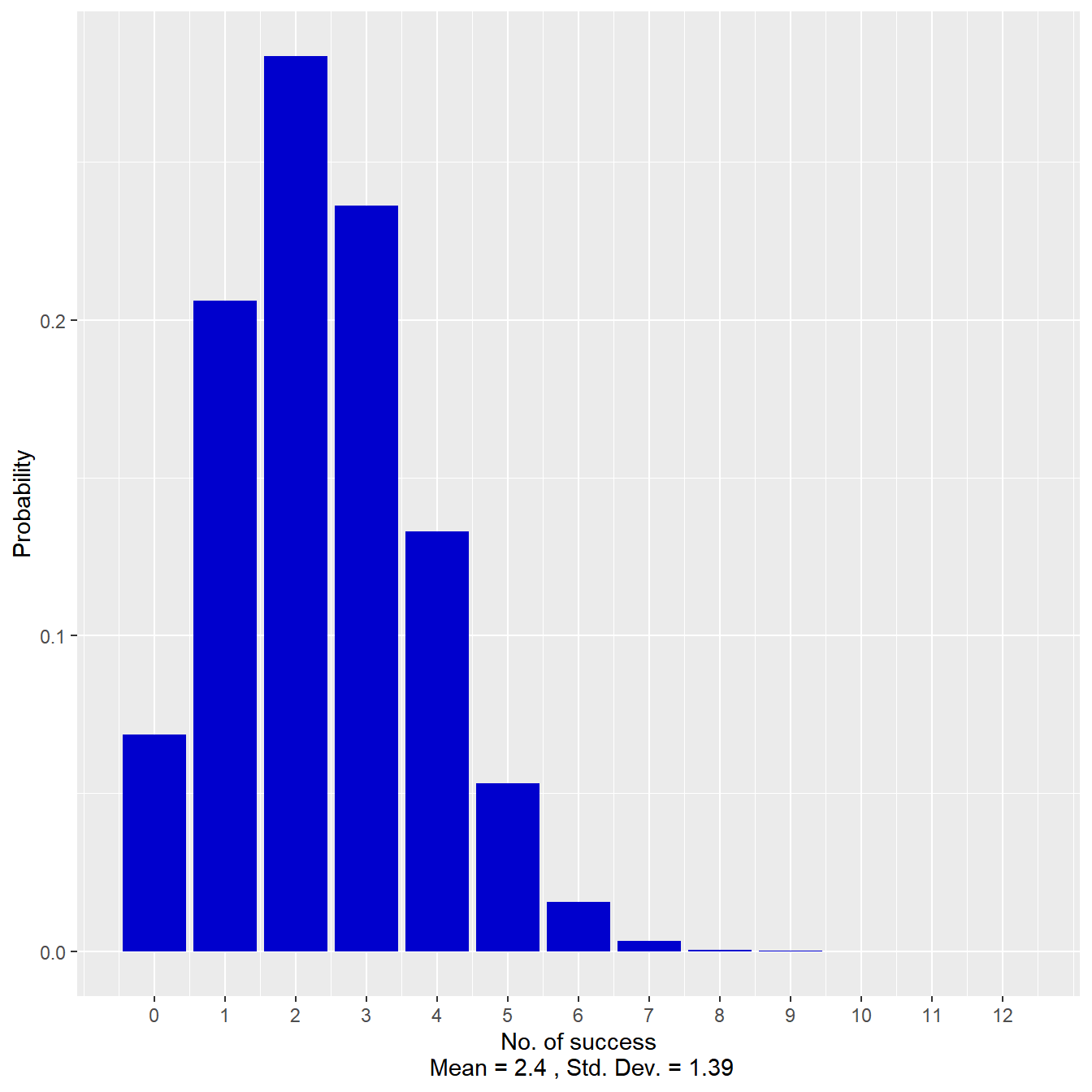What is the probability that fewer than 5 have no health insurance?

``vdist_binom_prob(12, 0.2, c(0, 4), 'interval')``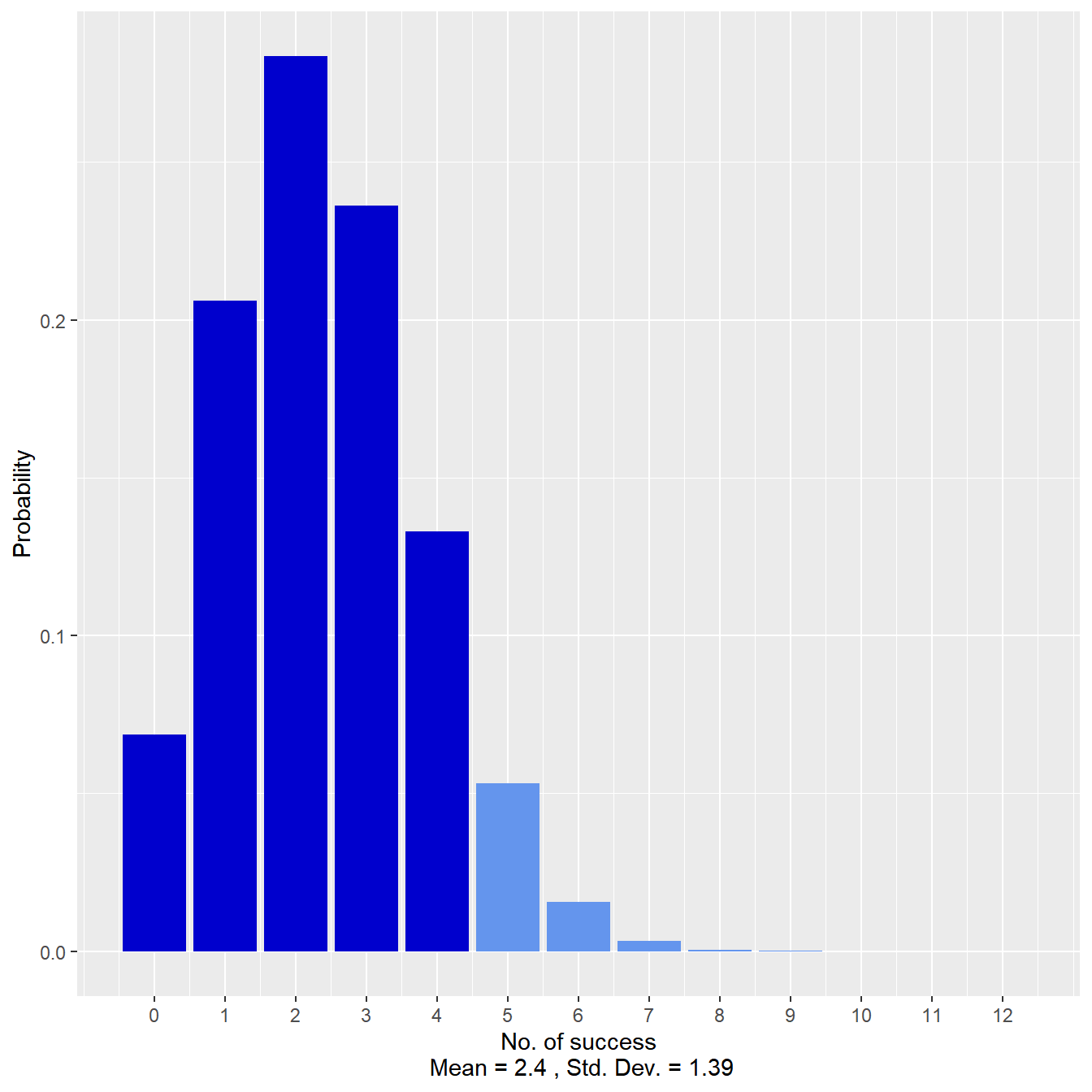## Chi Square Distribution

### Distribution Shape

Visualize how changes in degrees of freedom affect the shape of the chi square distribution.

``vdist_chisquare_plot(df = 5)``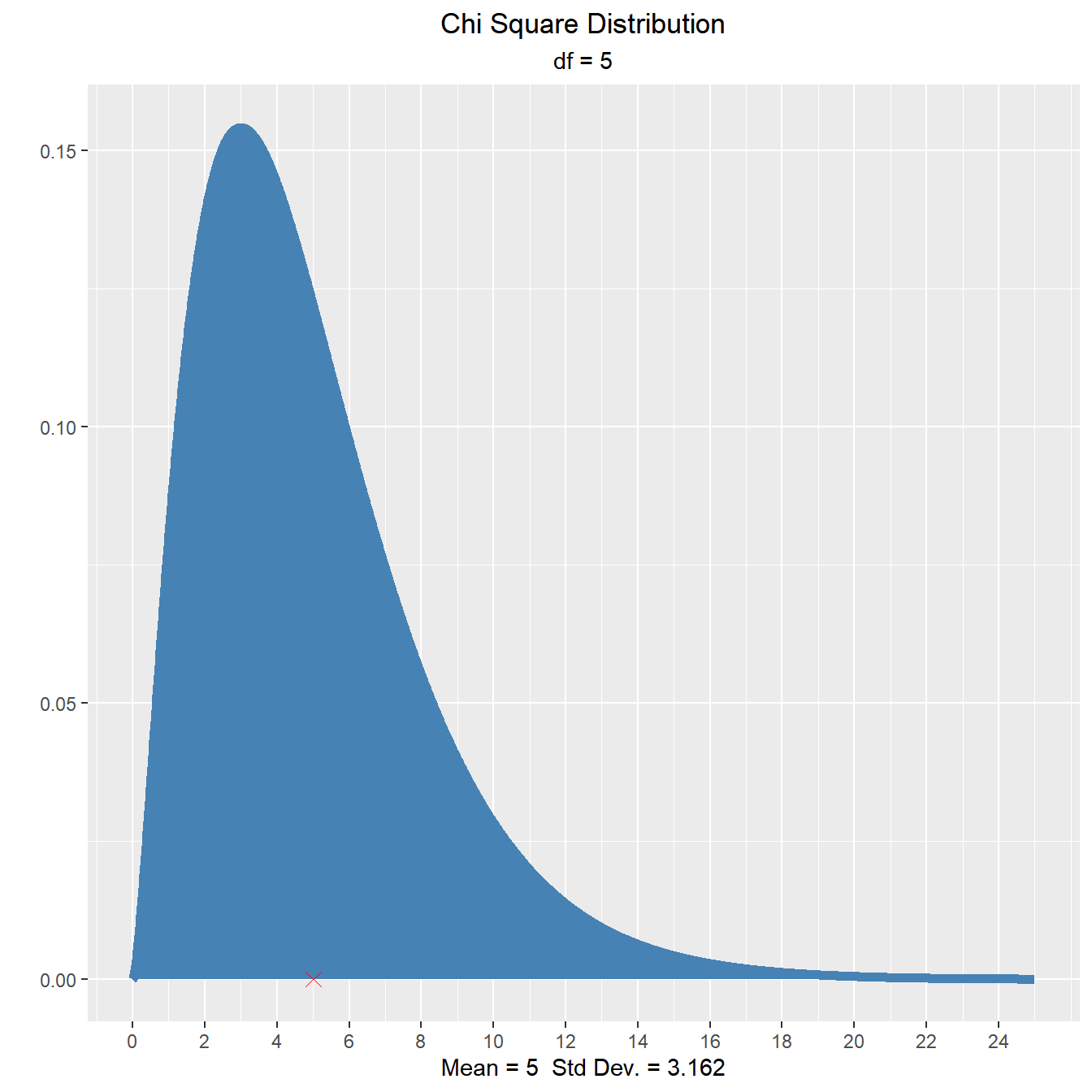``vdist_chisquare_plot(df = 5, normal = TRUE)``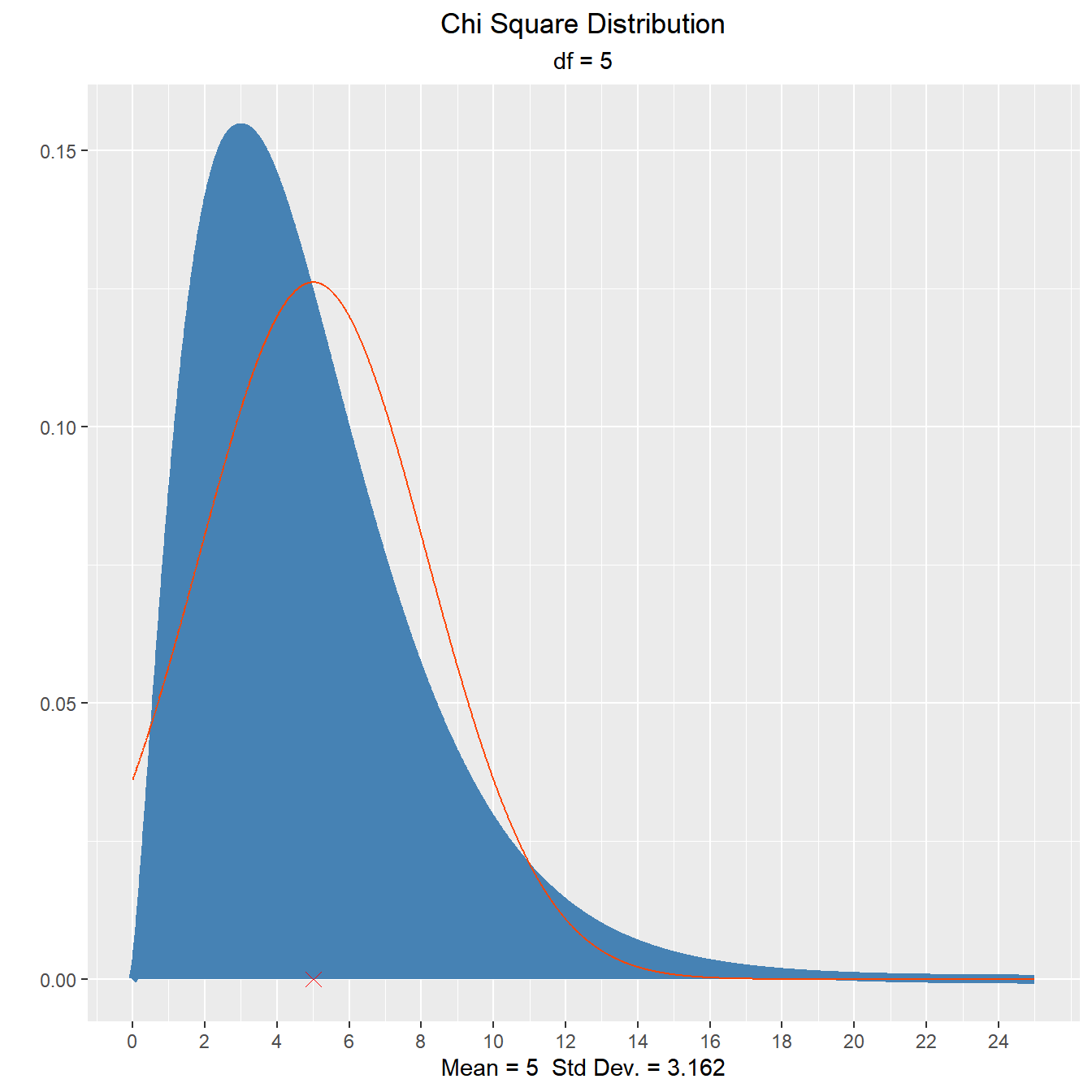### Percentiles

#### Calculate quantiles out of given probability

##### Input
• probs: a probability value
• df: degrees of freedom
• type: lower/upper tail

Let X be a chi-square random variable with 8 degrees of freedom. What is the upper fifth percentile?

``vdist_chisquare_perc(0.05, 8, 'upper')``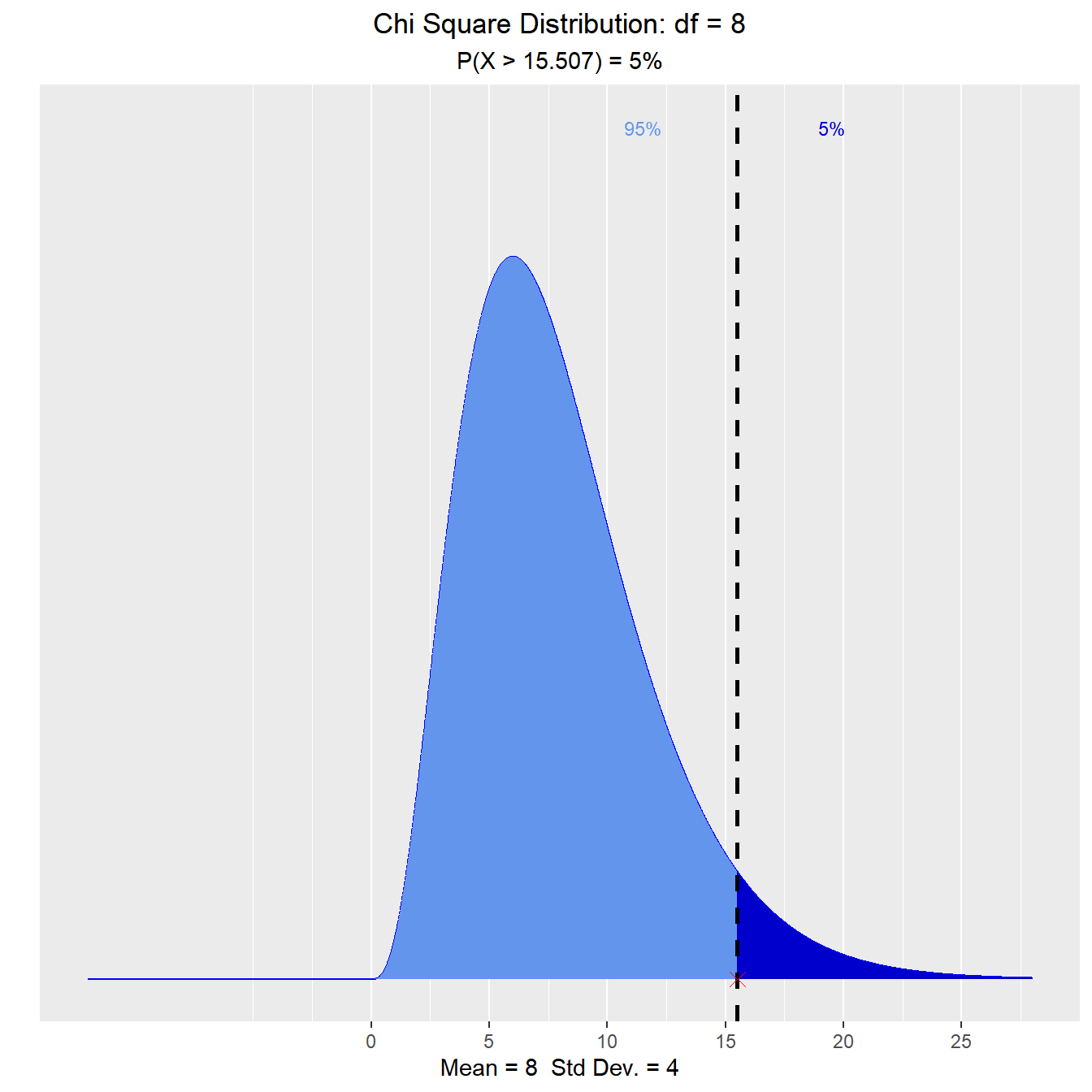What is the tenth percentile?

``vdist_chisquare_perc(0.10, 8, 'lower')``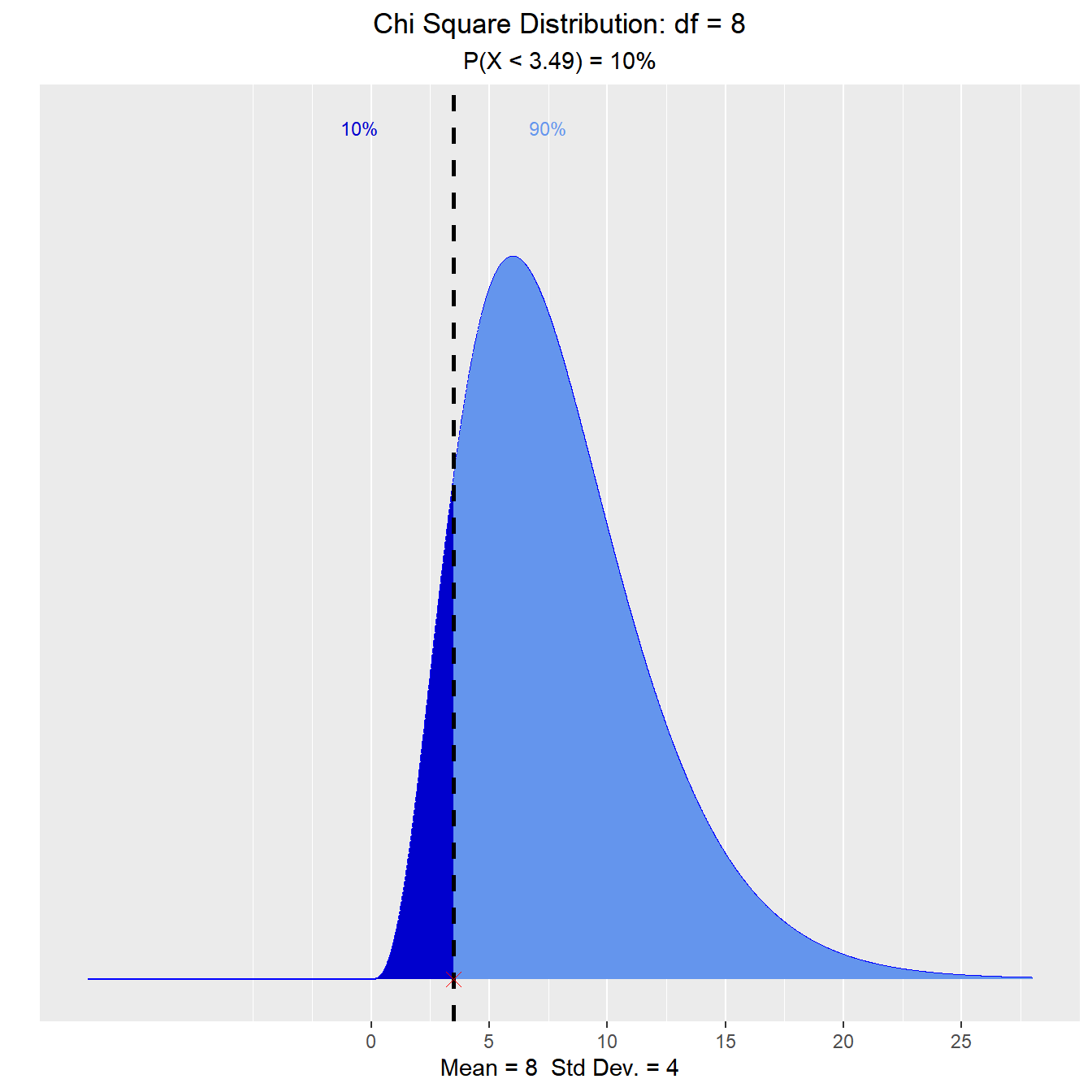### Probability

#### Calculate probability from a given quantile.

##### Input
• perc: a quantile value
• df: degrees of freedom
• type: lower/upper tail

What is the probability that a chi-square random variable with 12 degrees of freedom is greater than 8.79?

``vdist_chisquare_prob(8.79, 12, 'upper')``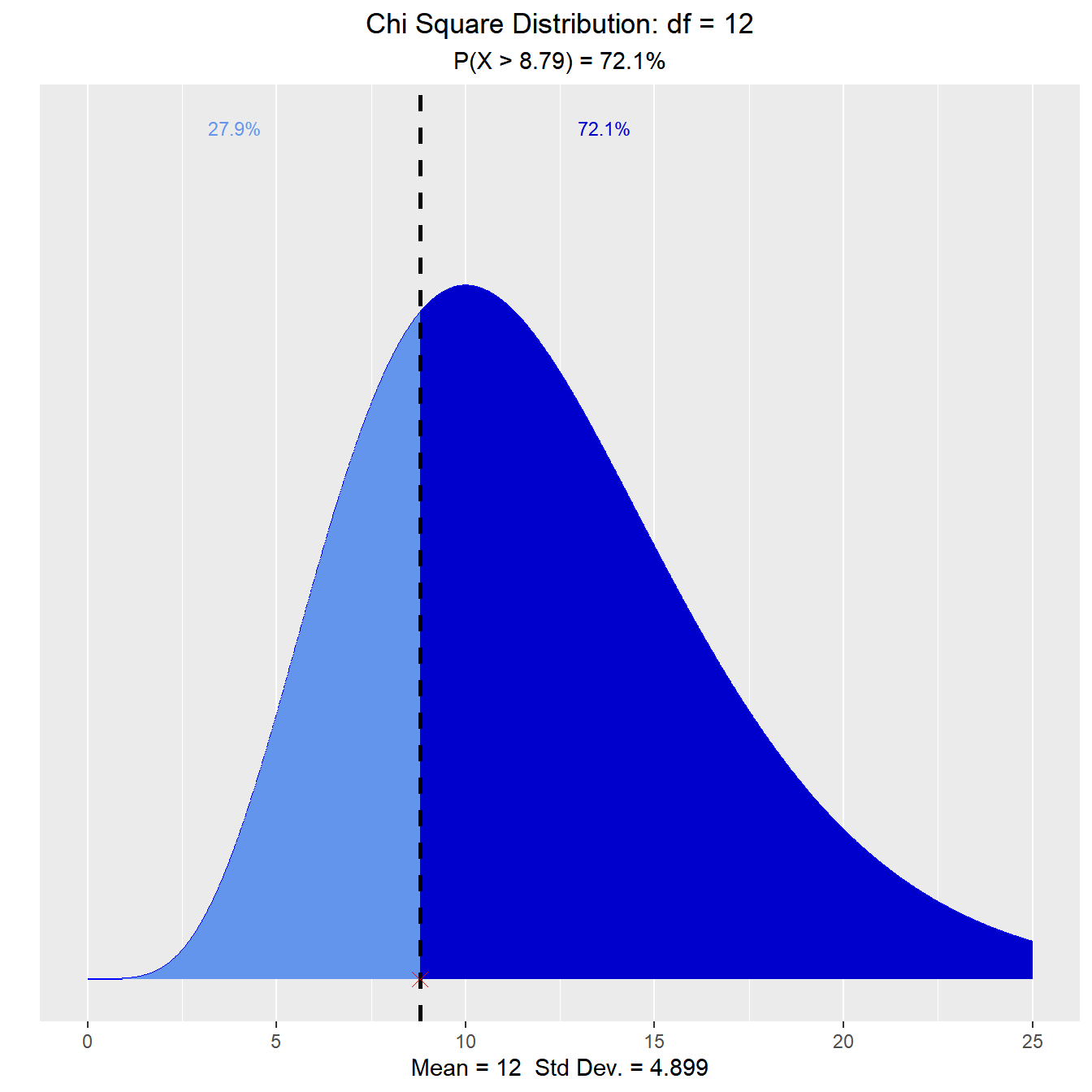What is the probability that a chi-square random variable with 12 degrees of freedom is greater than 8.62?

``vdist_chisquare_prob(8.62, 12, 'lower')``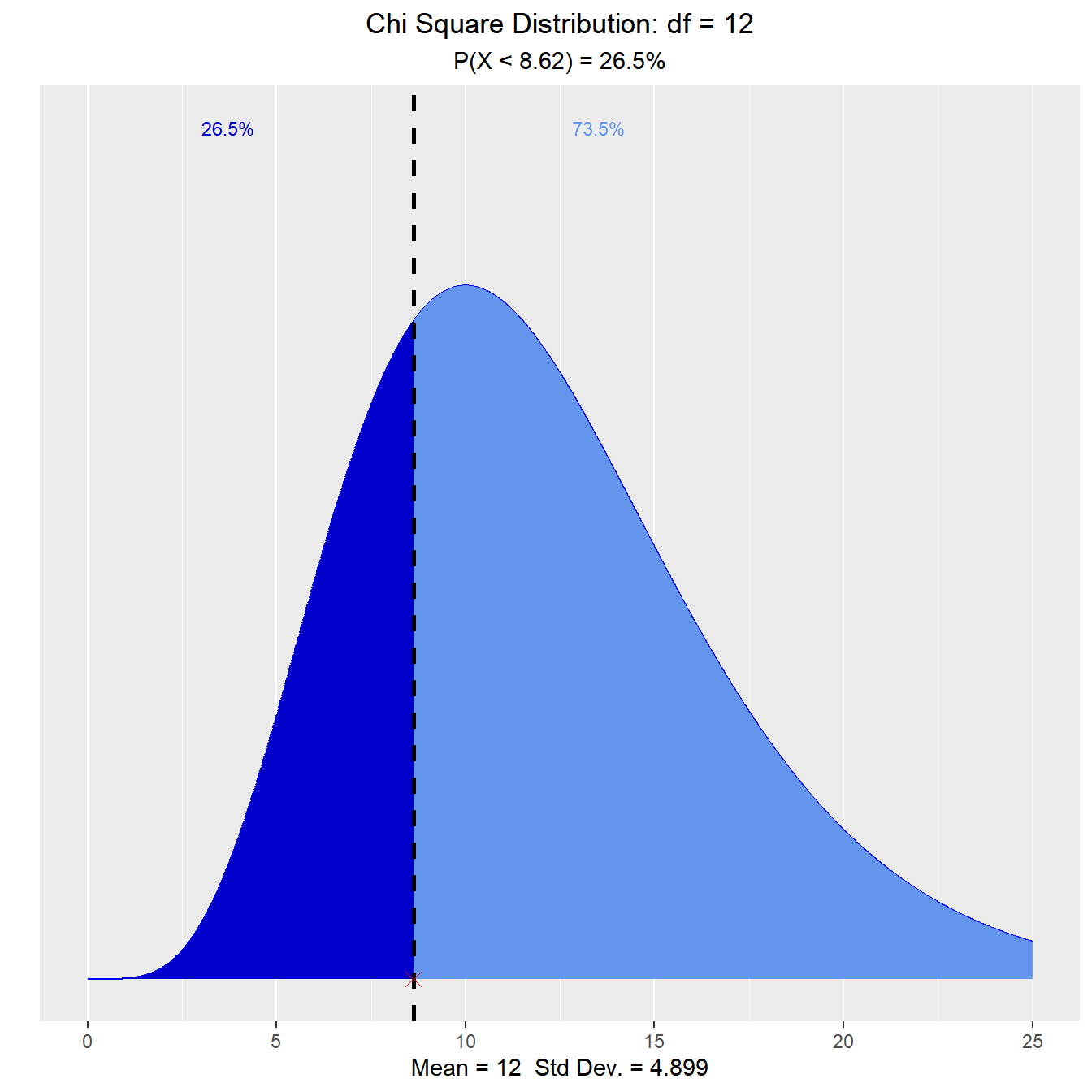## F Distribution

### Distribution Shape

Visualize how changes in degrees of freedom affect the shape of the F distribution.

``vdist_f_plot()``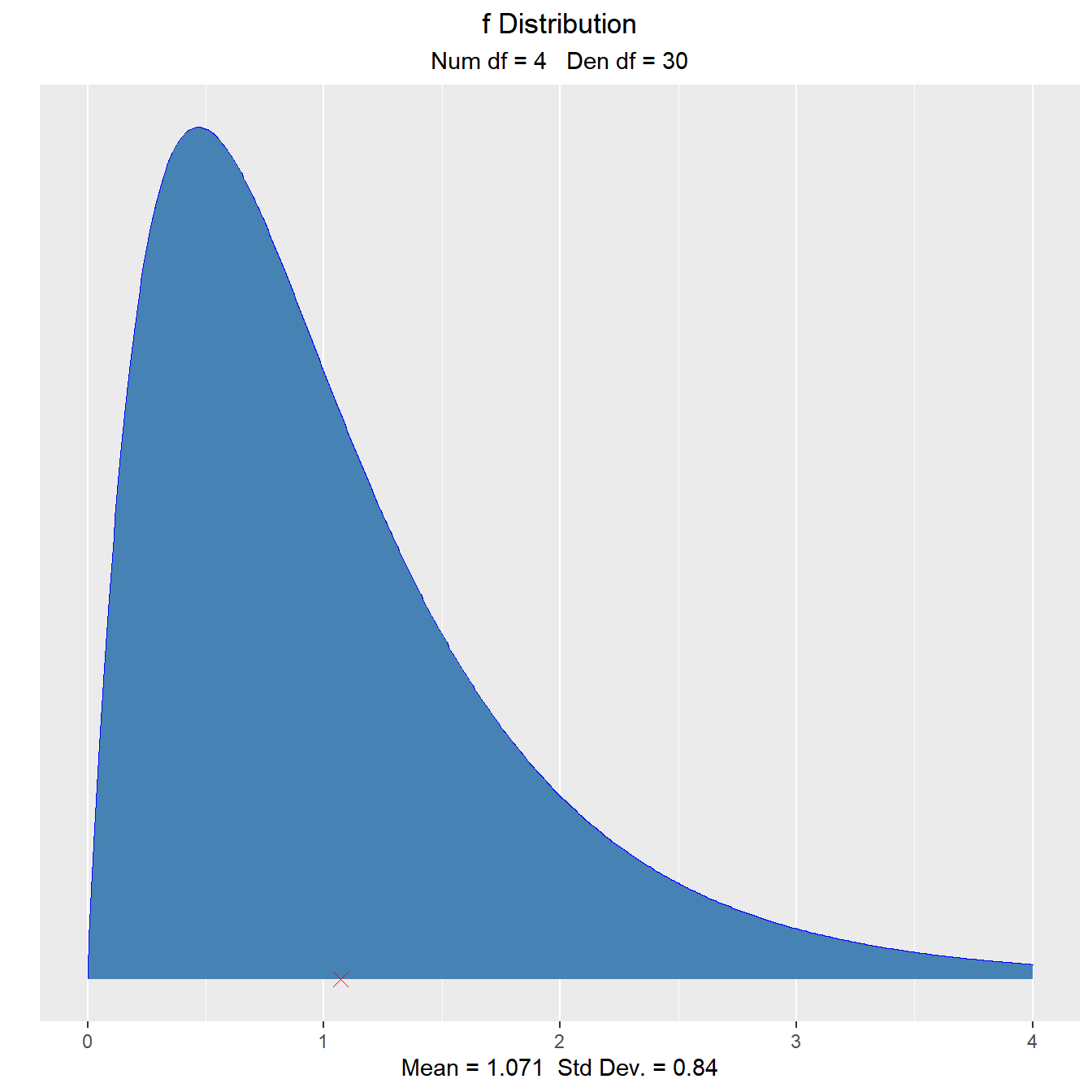``vdist_f_plot(6, 10, normal = TRUE)``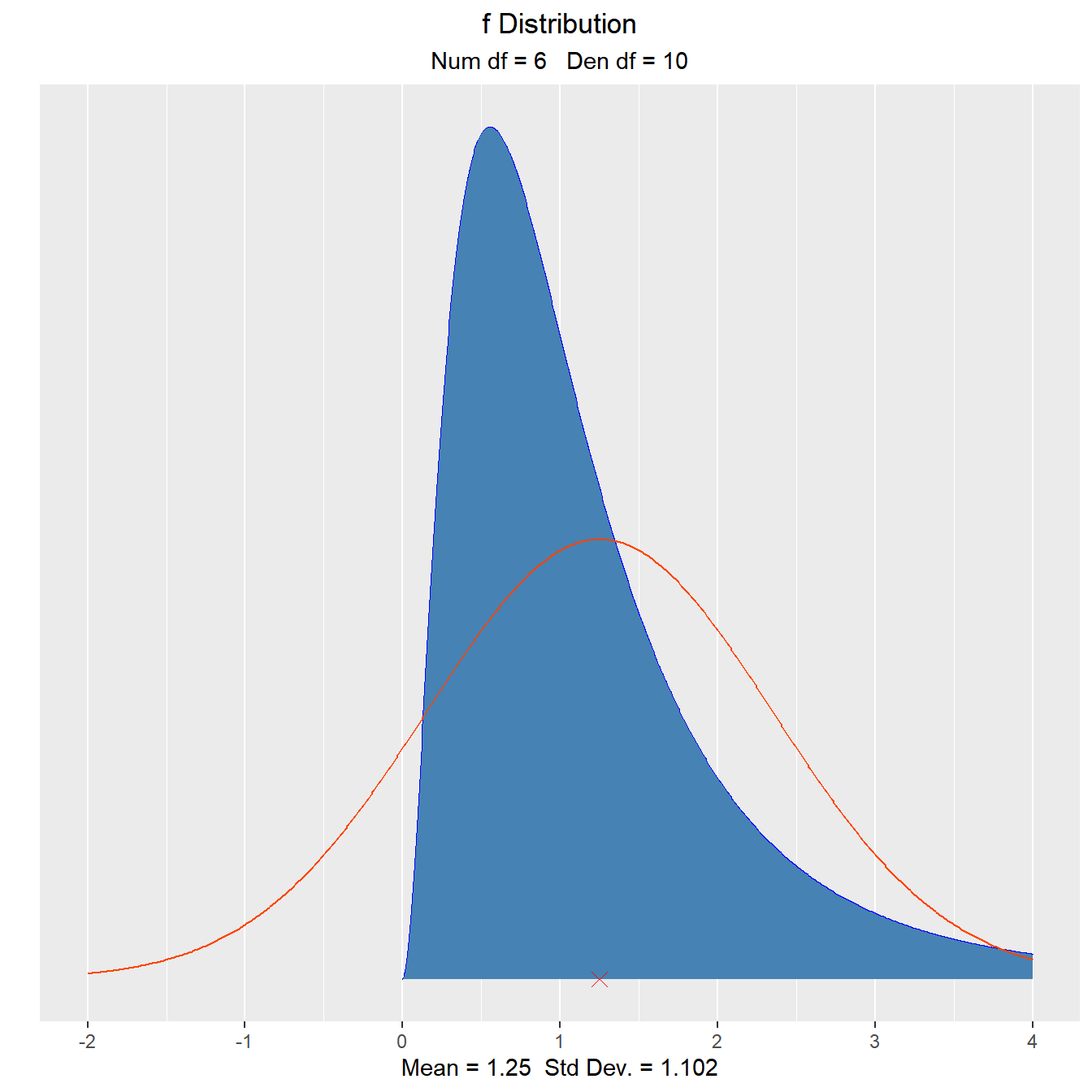### Percentiles

#### Calculate quantiles out of given probability

##### Input
• probs: a probability value
• num_df: nmerator degrees of freedom
• den_df: denominator degrees of freedom
• type: lower/upper tail

Let X be an F random variable with 4 numerator degrees of freedom and 5 denominator degrees of freedom. What is the upper twenth percentile?

``vdist_f_perc(0.20, 4, 5, 'upper')``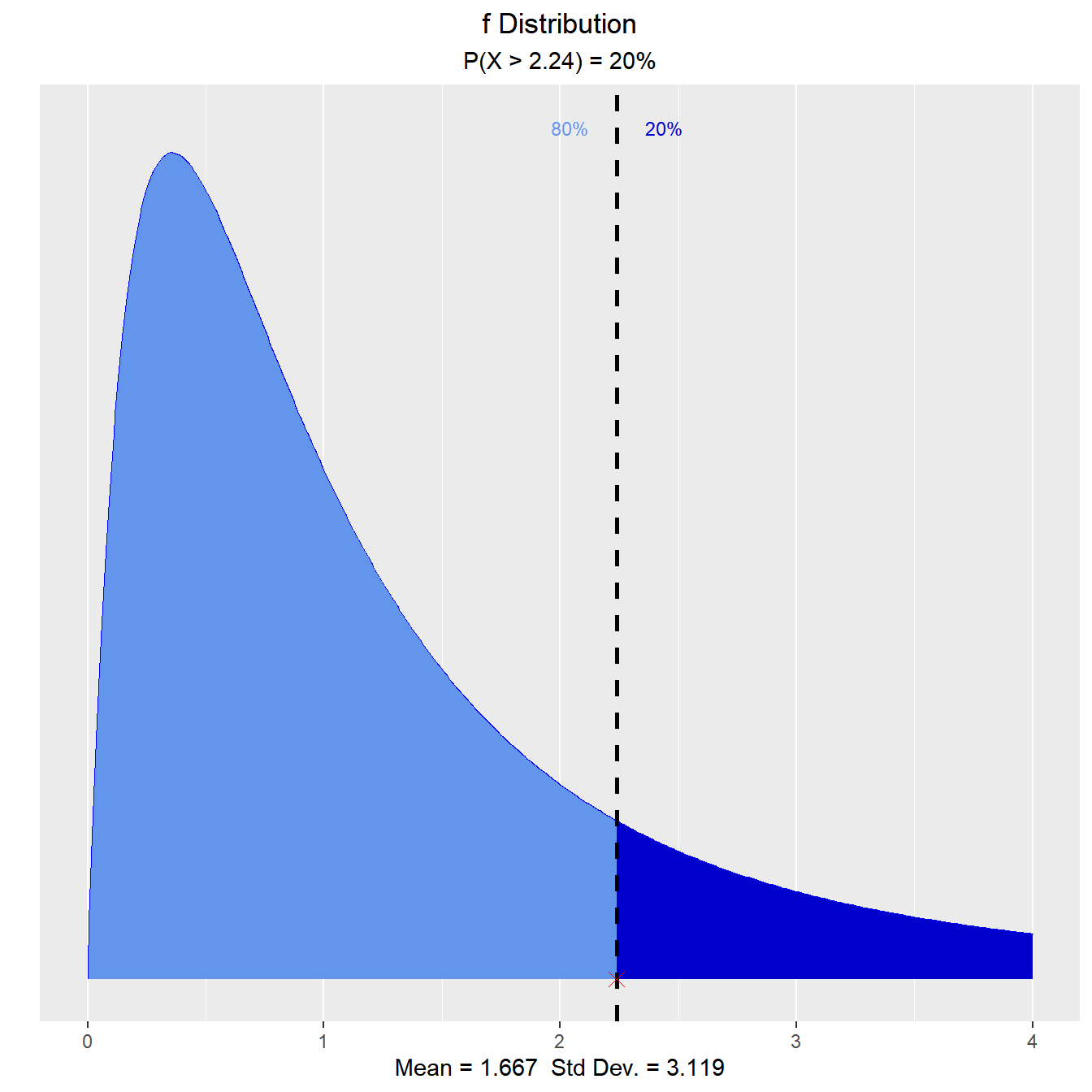What is the 35th percentile?

``vdist_f_perc(0.35, 4, 5, 'lower')``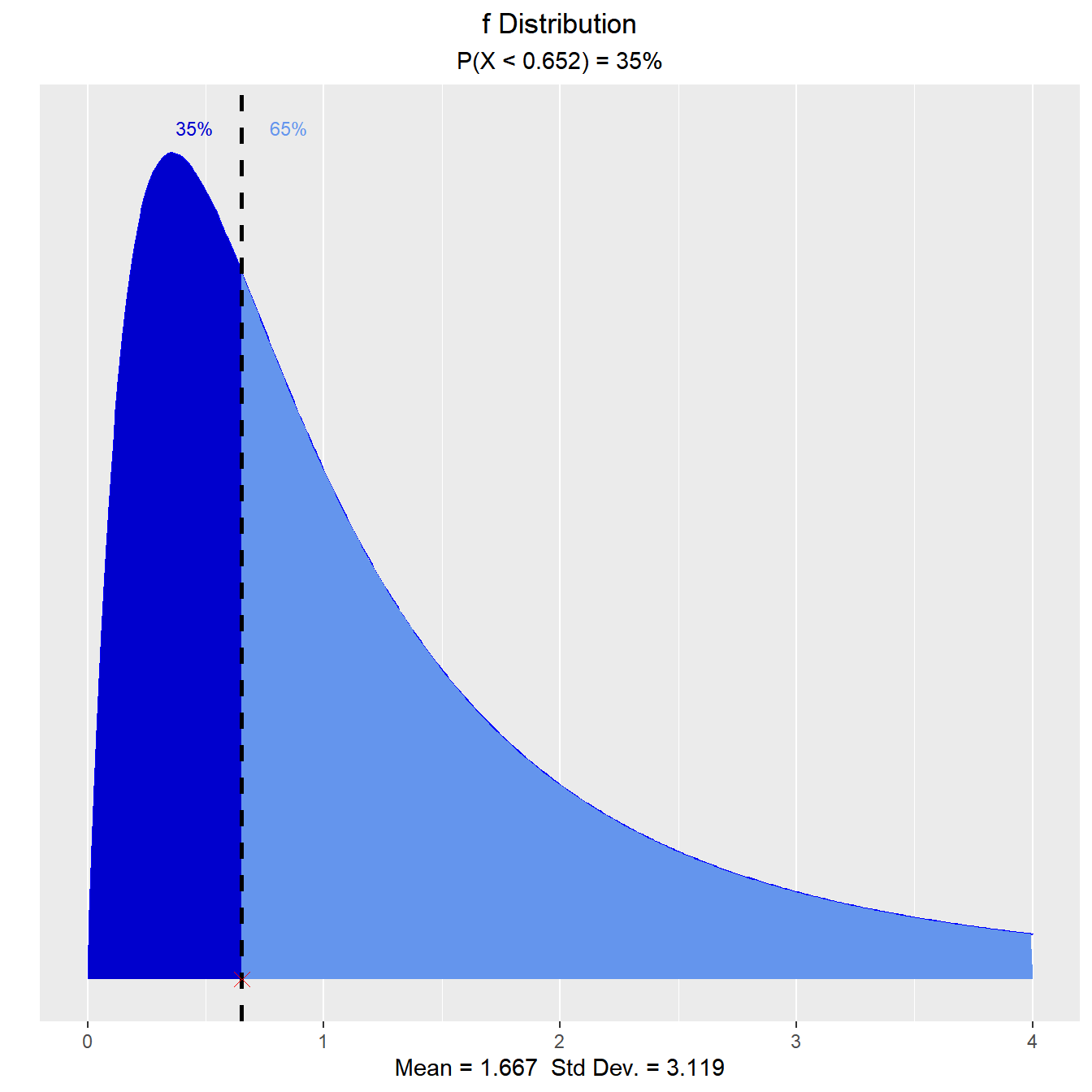### Probabilities

#### Calculate probability from a given quantile.

##### Input
• perc: a quantile value
• num_df: nmerator degrees of freedom
• den_df: denominator degrees of freedom
• type: lower/upper tail

What is the probability that an F random variable with 4 numerator degrees of freedom and 5 denominator degrees of freedom is greater than 3.89?

``vdist_f_prob(3.89, 4, 5, 'upper')``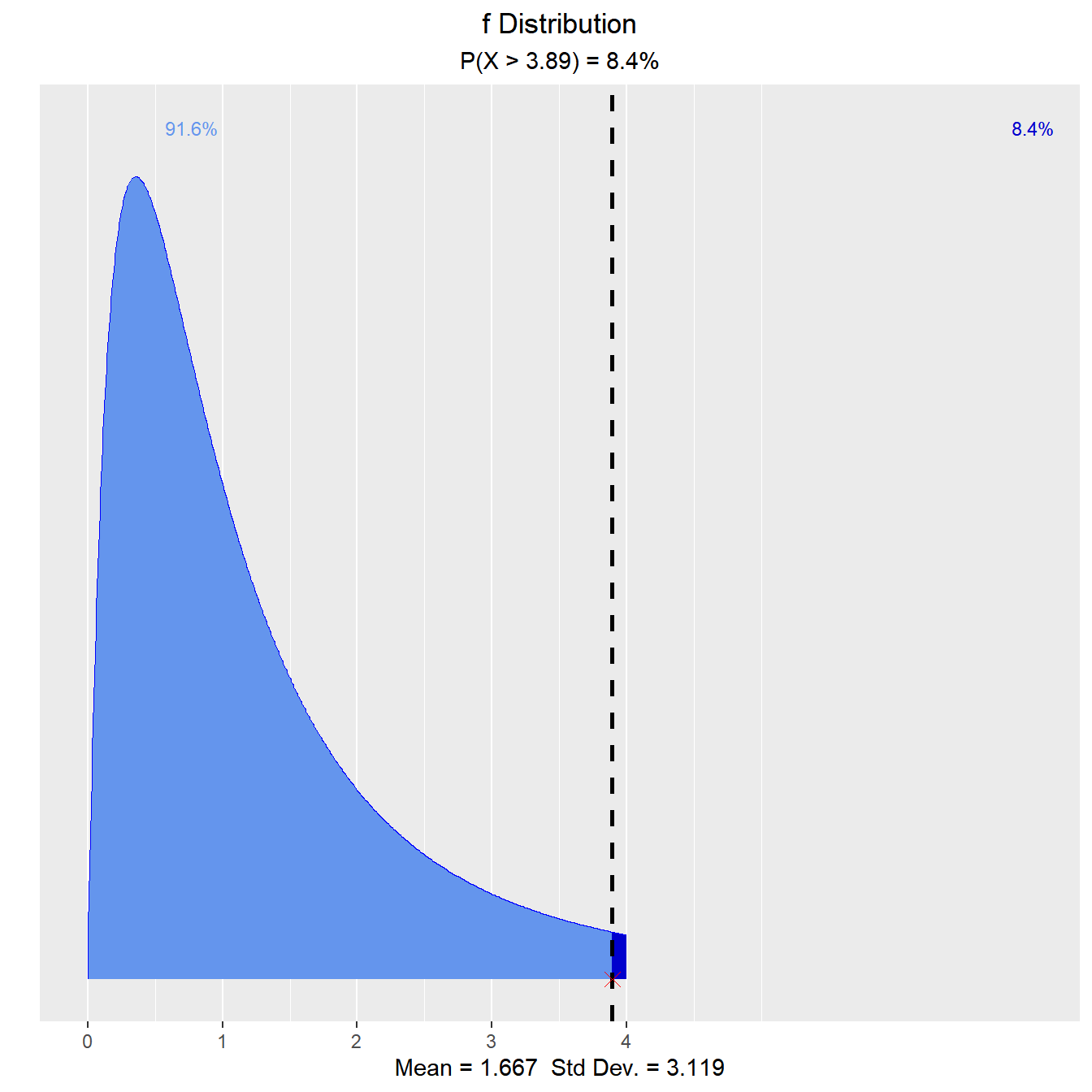What is the probability that an F random variable with 4 numerator degrees of freedom and 5 denominator degrees of freedom is less than 2.63?

``vdist_f_prob(2.63, 4, 5, 'lower')``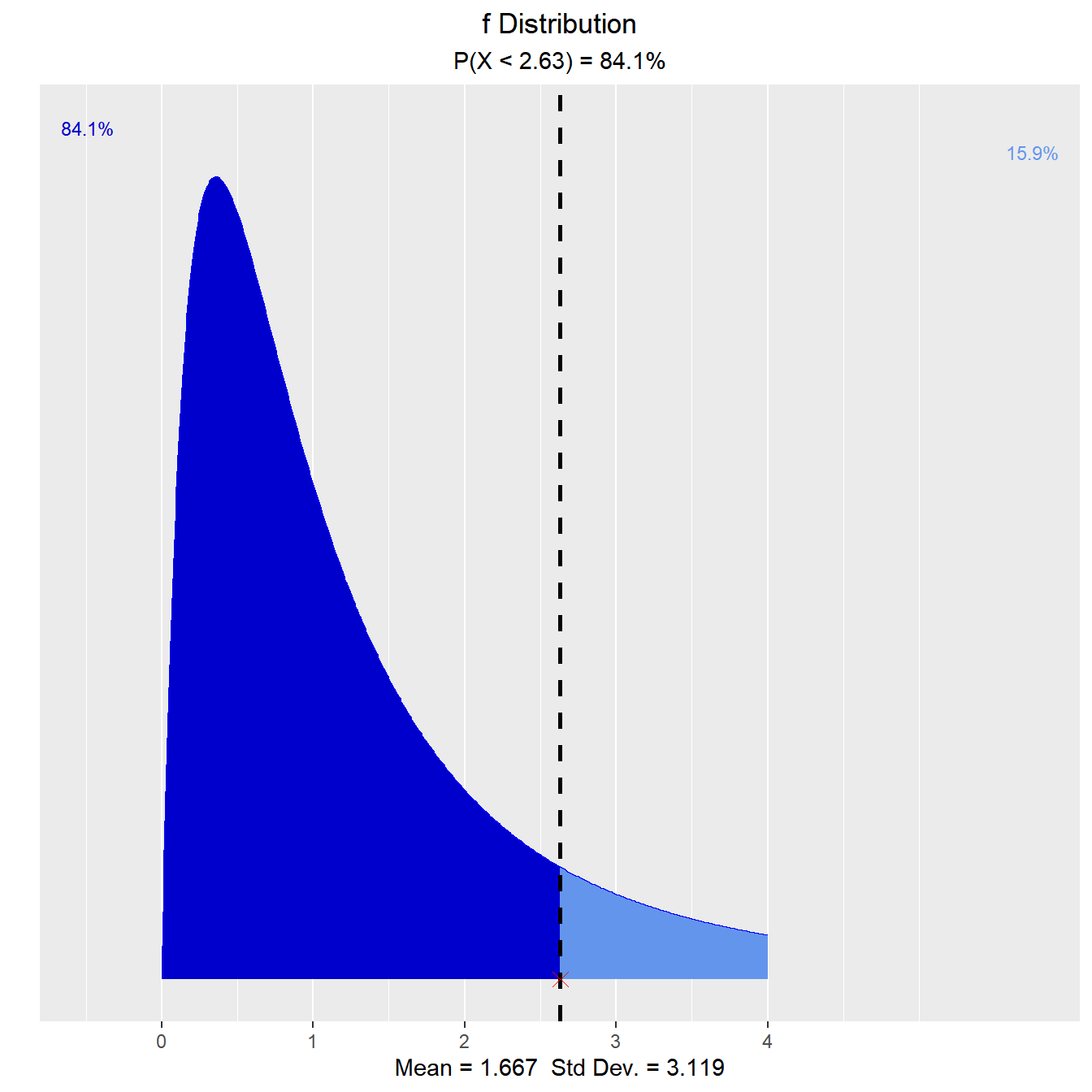## t Distribution

### Distribution Shape

Visualize how degrees of freedom affect the shape of t distribution.

``vdist_t_plot(df = 8)``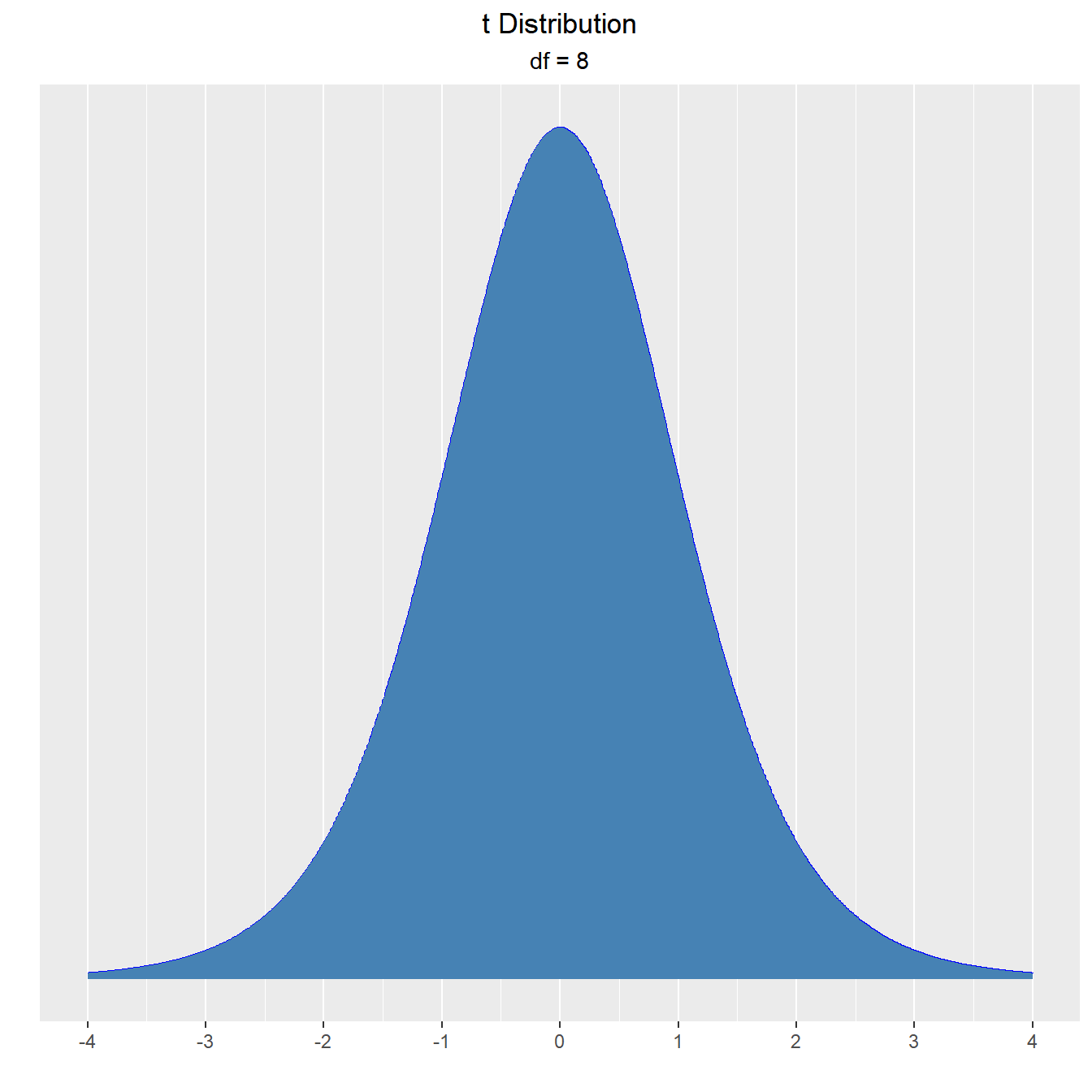### Percentiles

#### Calculate quantiles out of given probability

##### Input
• probs: a probability value
• df: degrees of freedom
• type: lower/upper/both tail

What is the upper fifteenth percentile?

``vdist_t_perc(0.15, 8, 'upper')``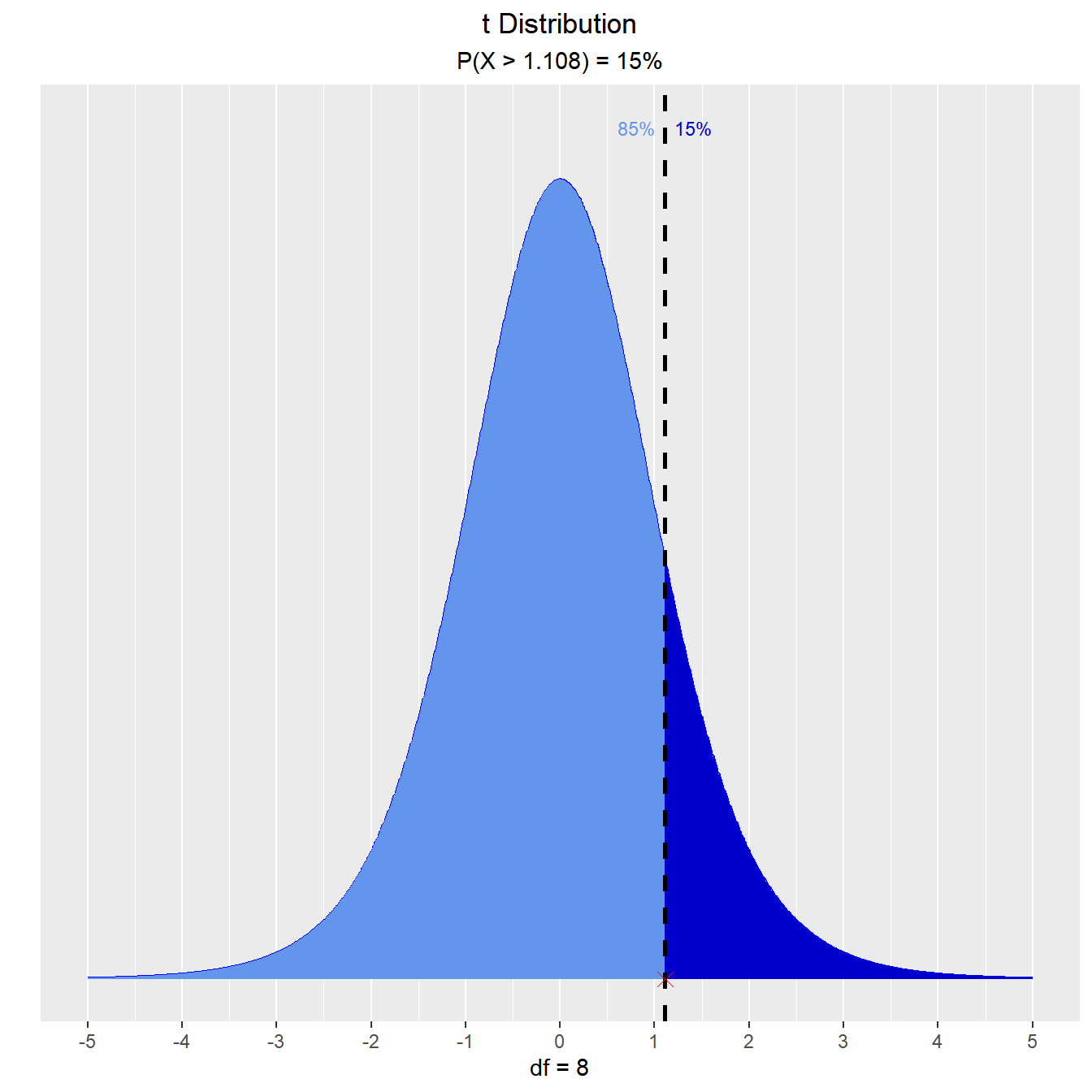What is the eleventh percentile?

``vdist_t_perc(0.11, 8, 'lower')``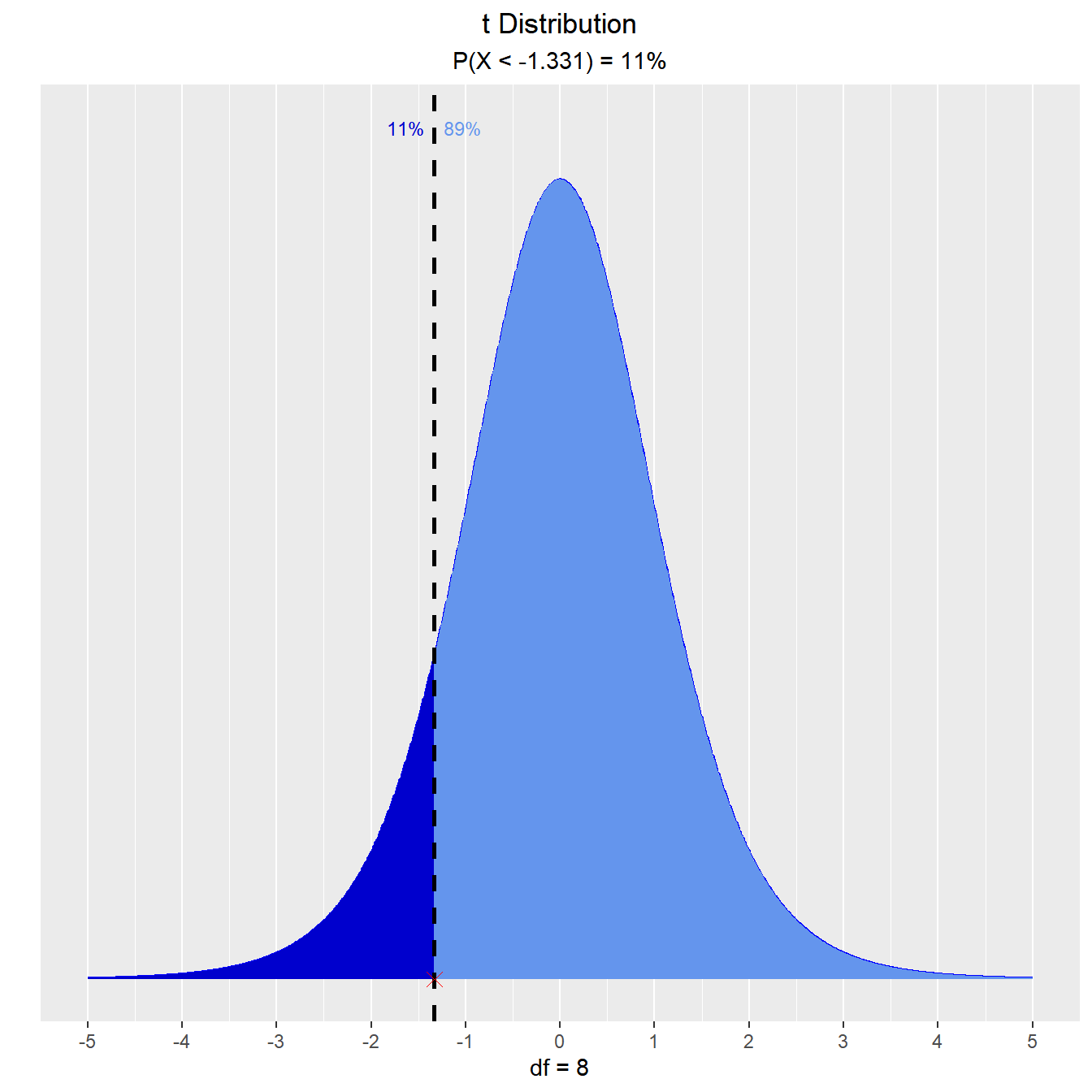What is the area of the curve that has 95% of the t values?

``vdist_t_perc(0.8, 8, 'both')``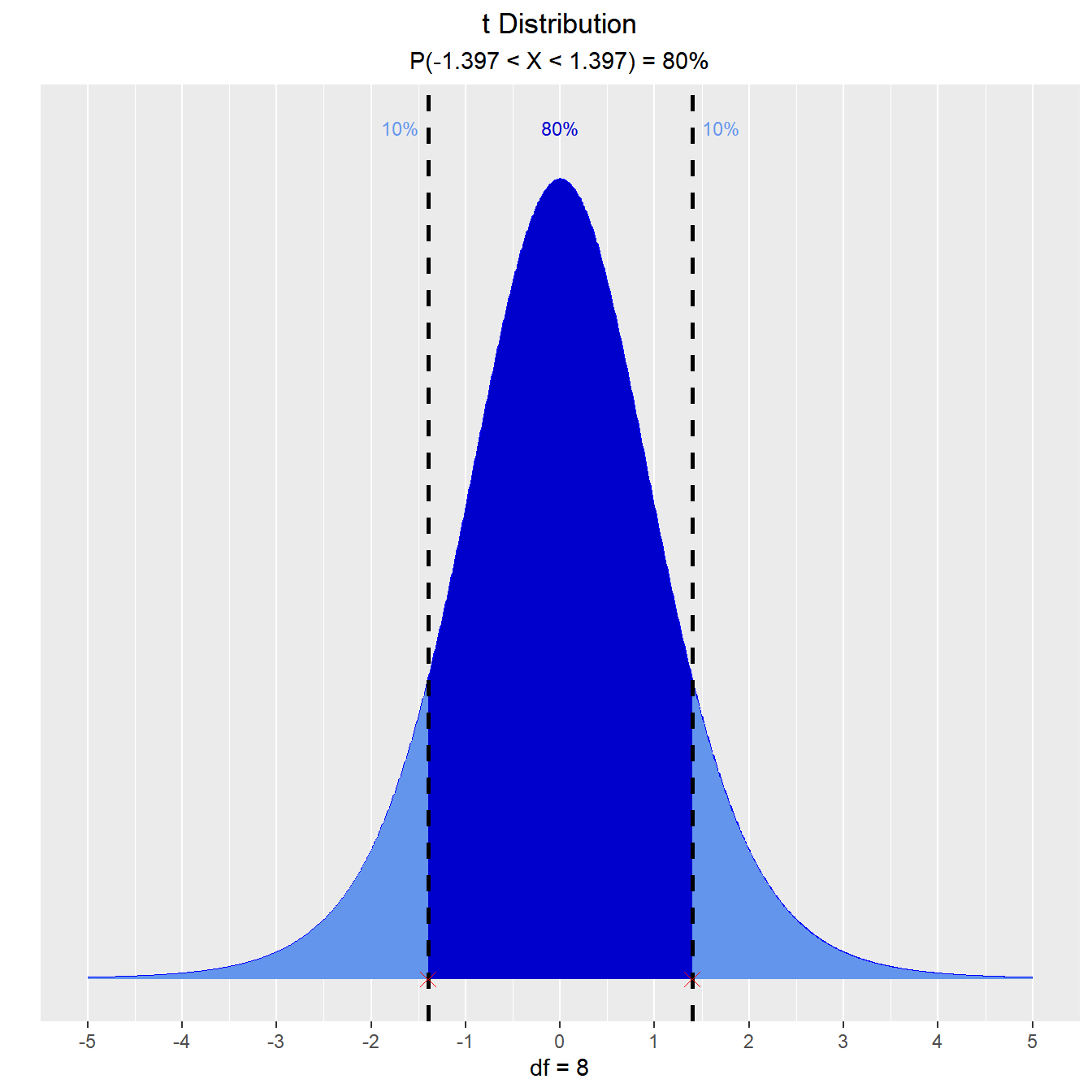### Probabilities

#### Calculate probability from a given quantile.

##### Input
• perc: a quantile value
• df: degrees of freedom
• type: lower/upper/interval/both tail

Let T follow a t-distribution with r = 6 df.

What is the probability that the value of T is less than 2?

``vdist_t_prob(2, 6, 'lower')``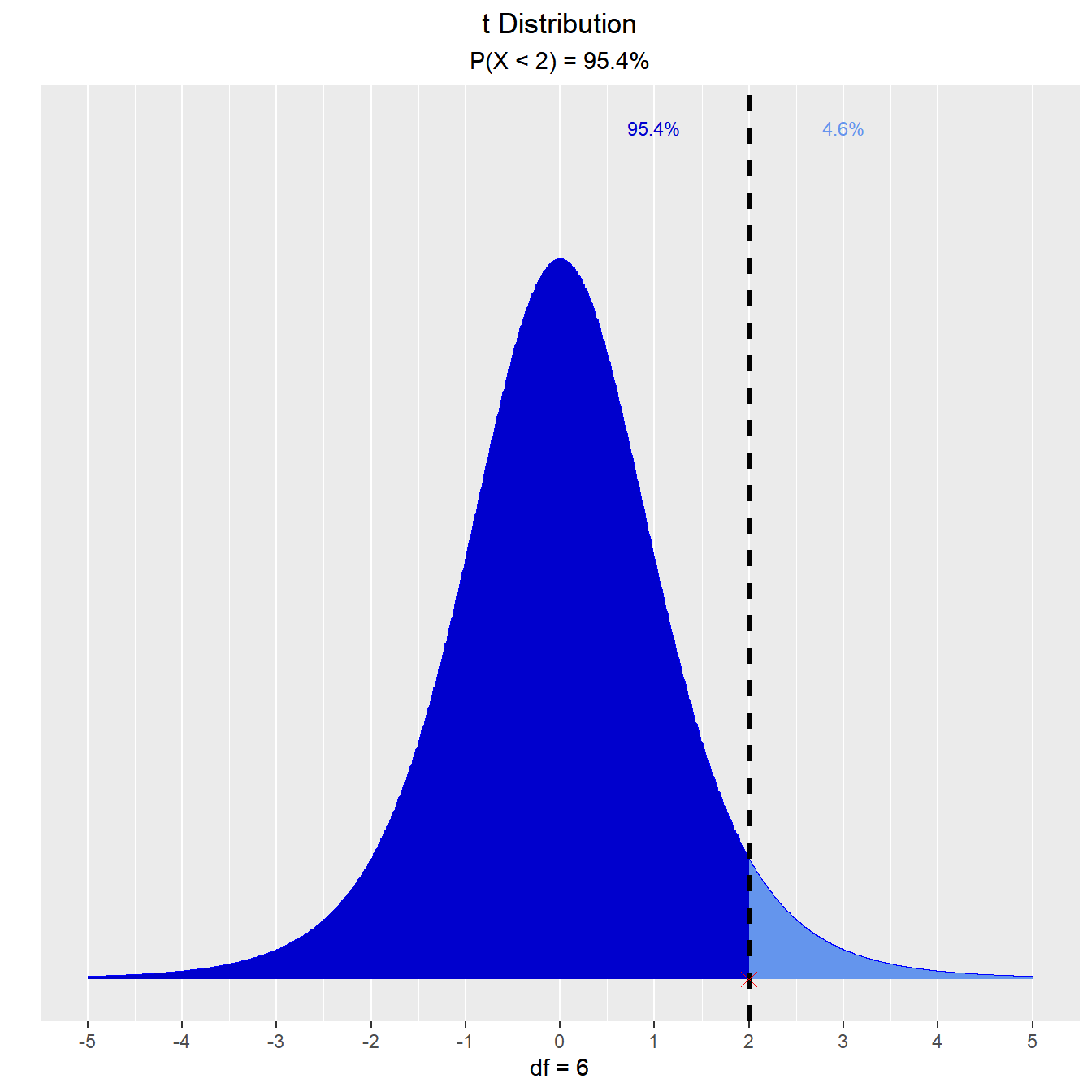What is the probability that the value of T is greater than 2?

``vdist_t_prob(2, 6, 'upper')``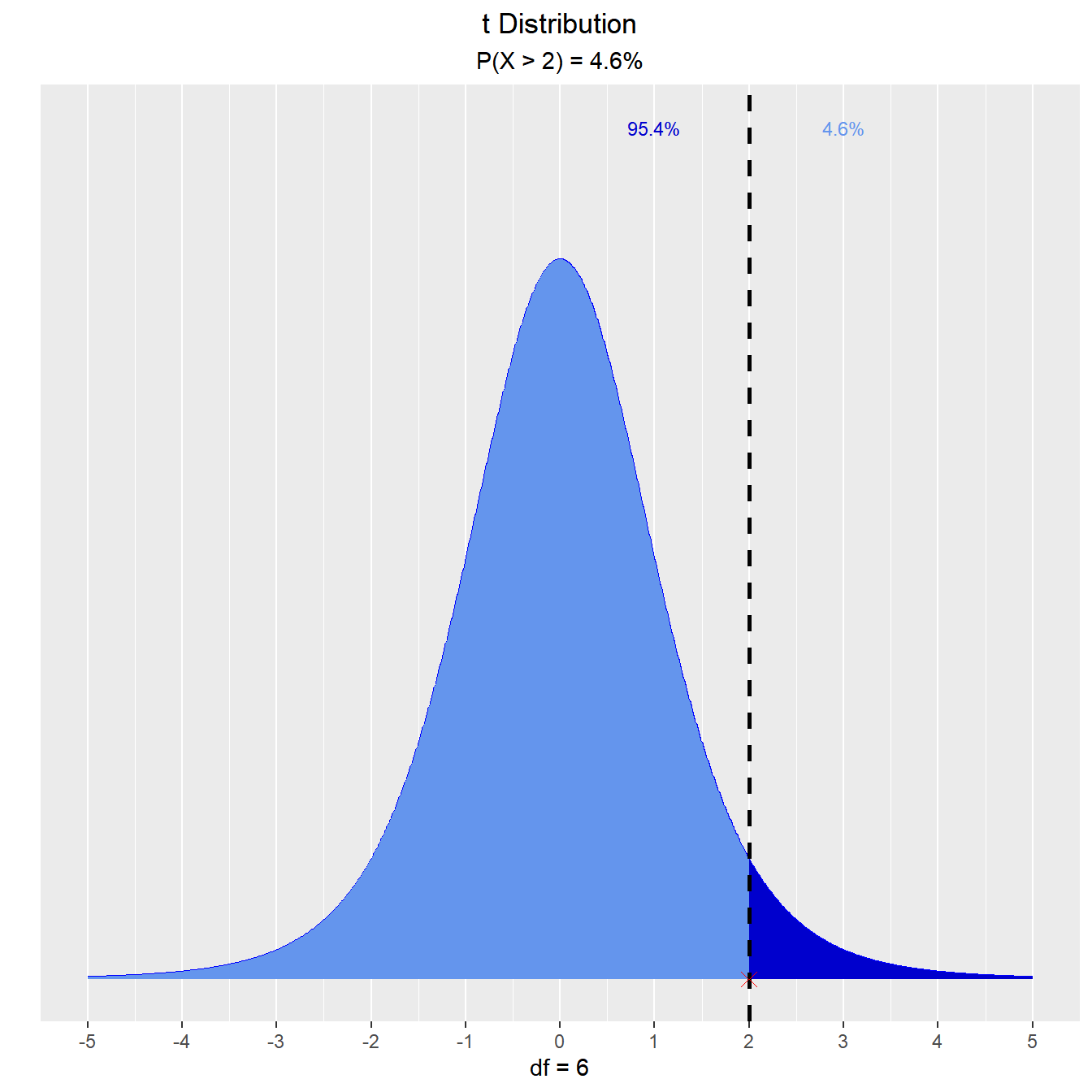What is the probability that the value of T is between -2 and 2?

``vdist_t_prob(2, 6, 'both')``What is the probability that the absolute value of T is greater than 2?

``vdist_t_prob(2, 6, 'interval')``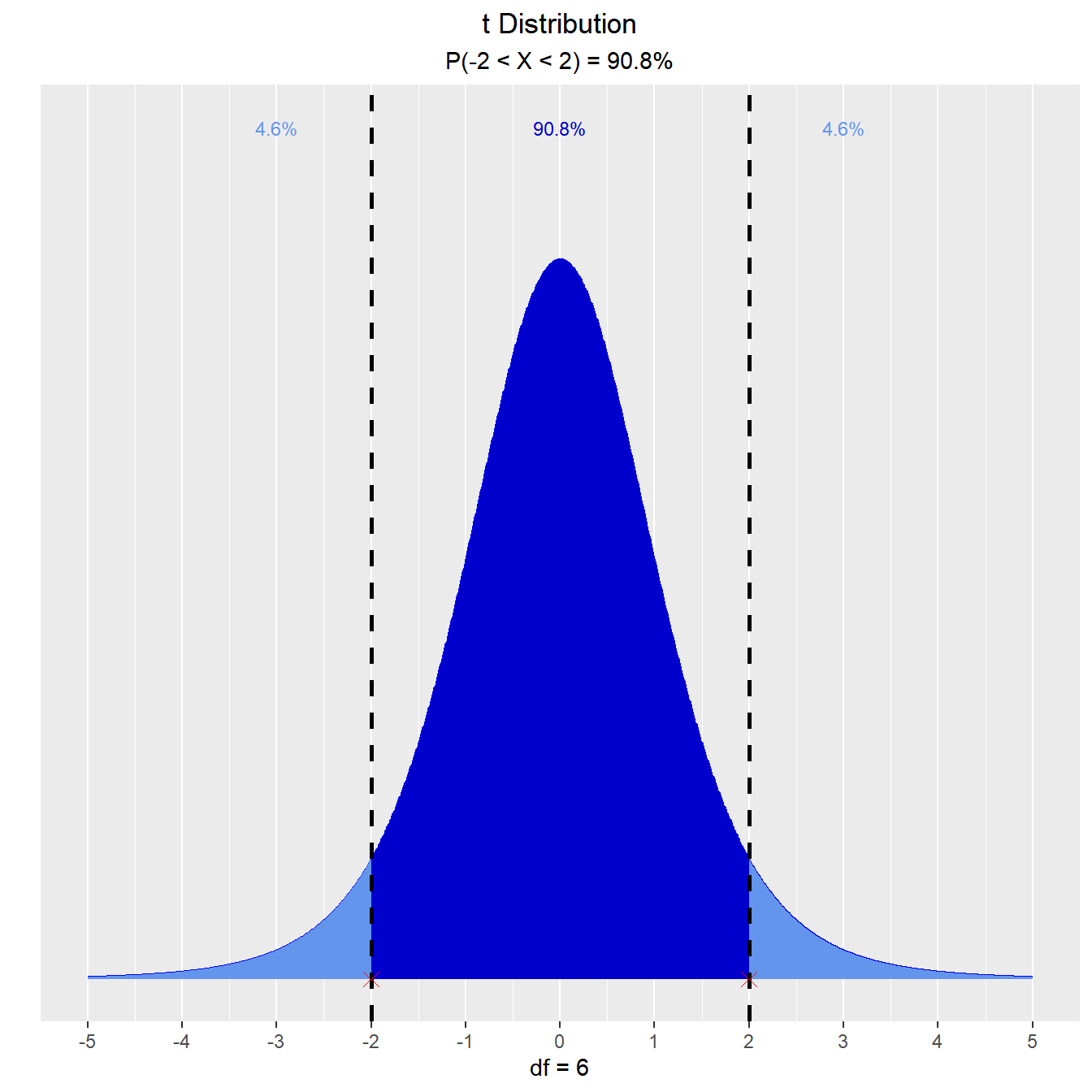## Learning More

The vistributions website includes comprehensive documentation on using the package, including the following article that gives a brief introduction to vistributions:

## Feedback

vistributions has been on CRAN for a few months now while we were fixing bugs and making the API stable. All feedback is welcome. Issues (bugs and feature requests) can be posted to github tracker. For help with code or other related questions, feel free to reach out to us at .